Learn to click the perfect selfie Learn to click the perfect selfie

# Concise Selina Solutions for Class 9 Maths Chapter 22- Trigonometrical Ratios

The Selina Solutions for the questions given in Chapter 22, Trigonometrical Ratios, of the Class 9 Selina textbooks are available here. In this chapter students learn about the topic of Trigonometrical Ratios of a triangle, dealing with the relations of sides and angles of right-angled triangles. Students can easily score full marks in the exams by solving all the questions present in the Selina textbook.

The Class 9 Selina Solutions Maths is very easy to understand. These solutions cover all the exercise questions included in the book and are according to the syllabus prescribed by the ICSE or CISCE. Here, the PDF of the Class 9 Maths Chapter 22 Selina Solutions is available, which can be downloaded as well as viewed online. Students can also avail the Selina Solutions and download it for free to practice offline as well.

### Download PDF of Selina Solutions for Class 9 Maths Chapter 22:-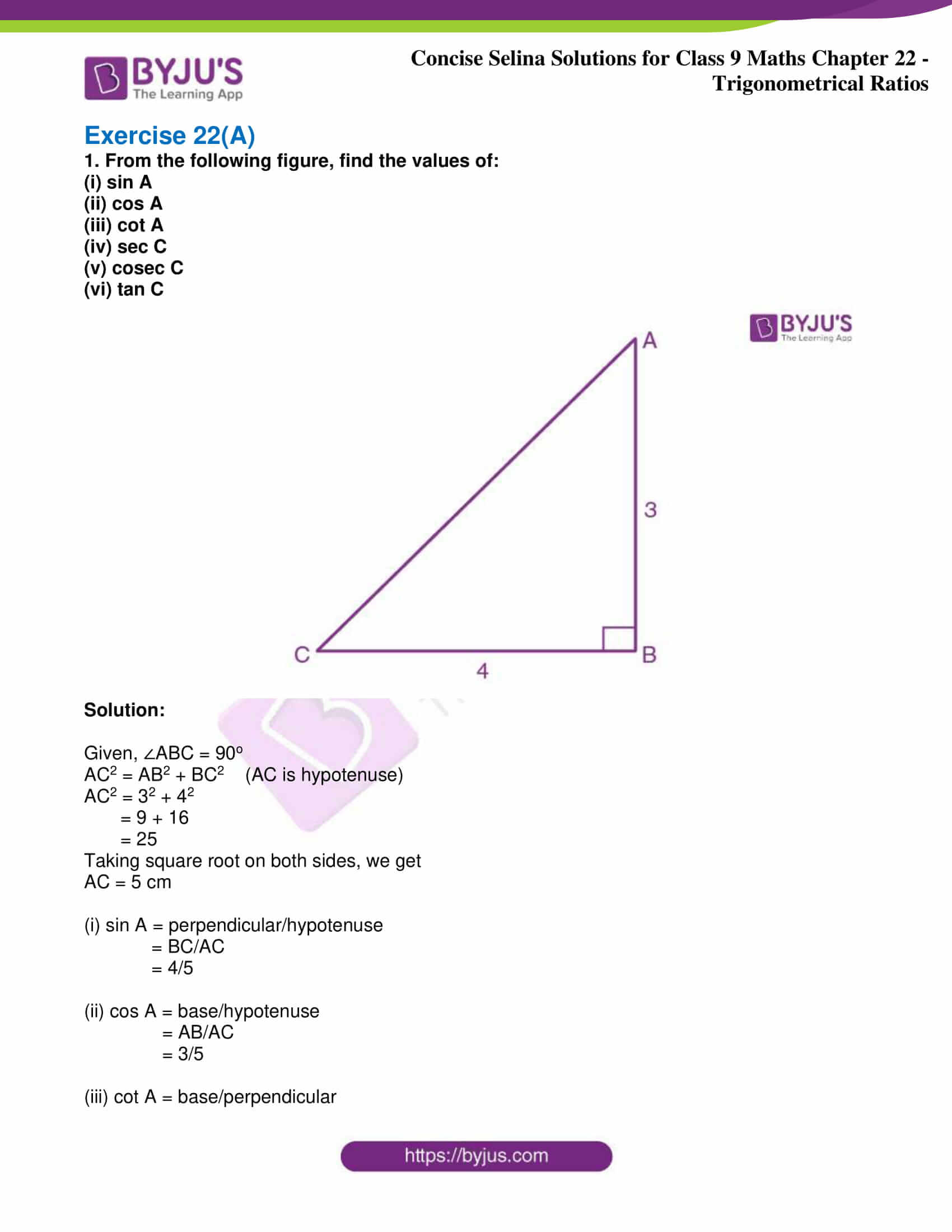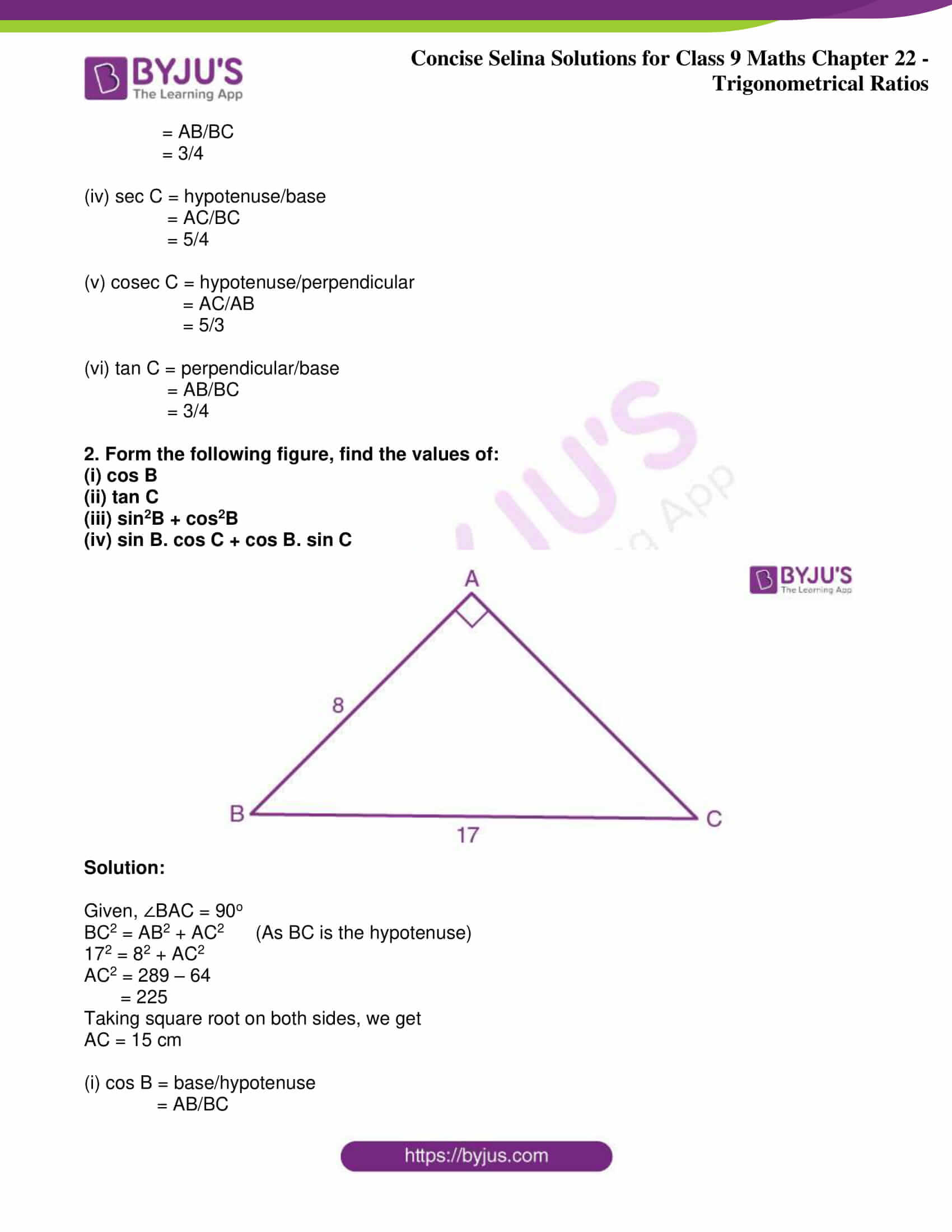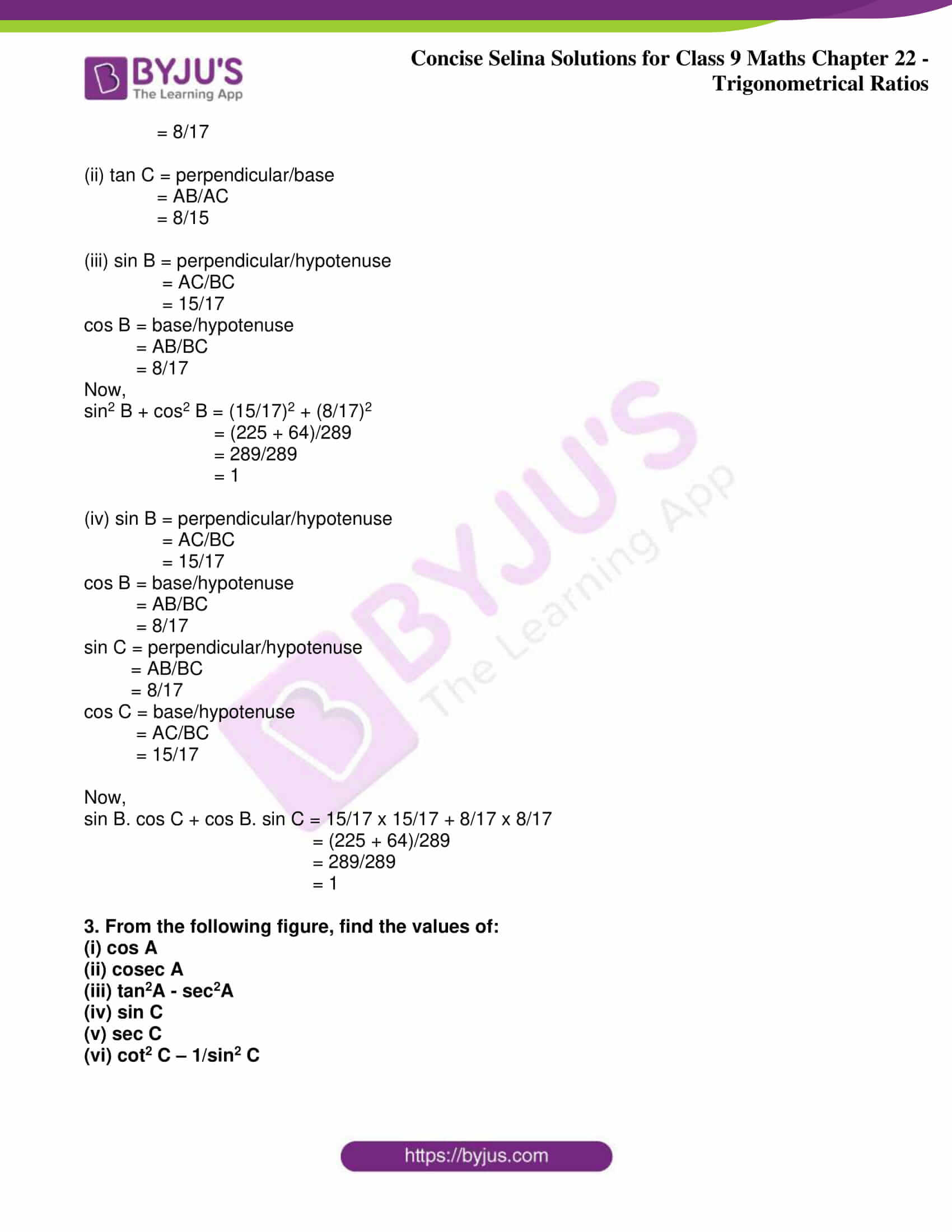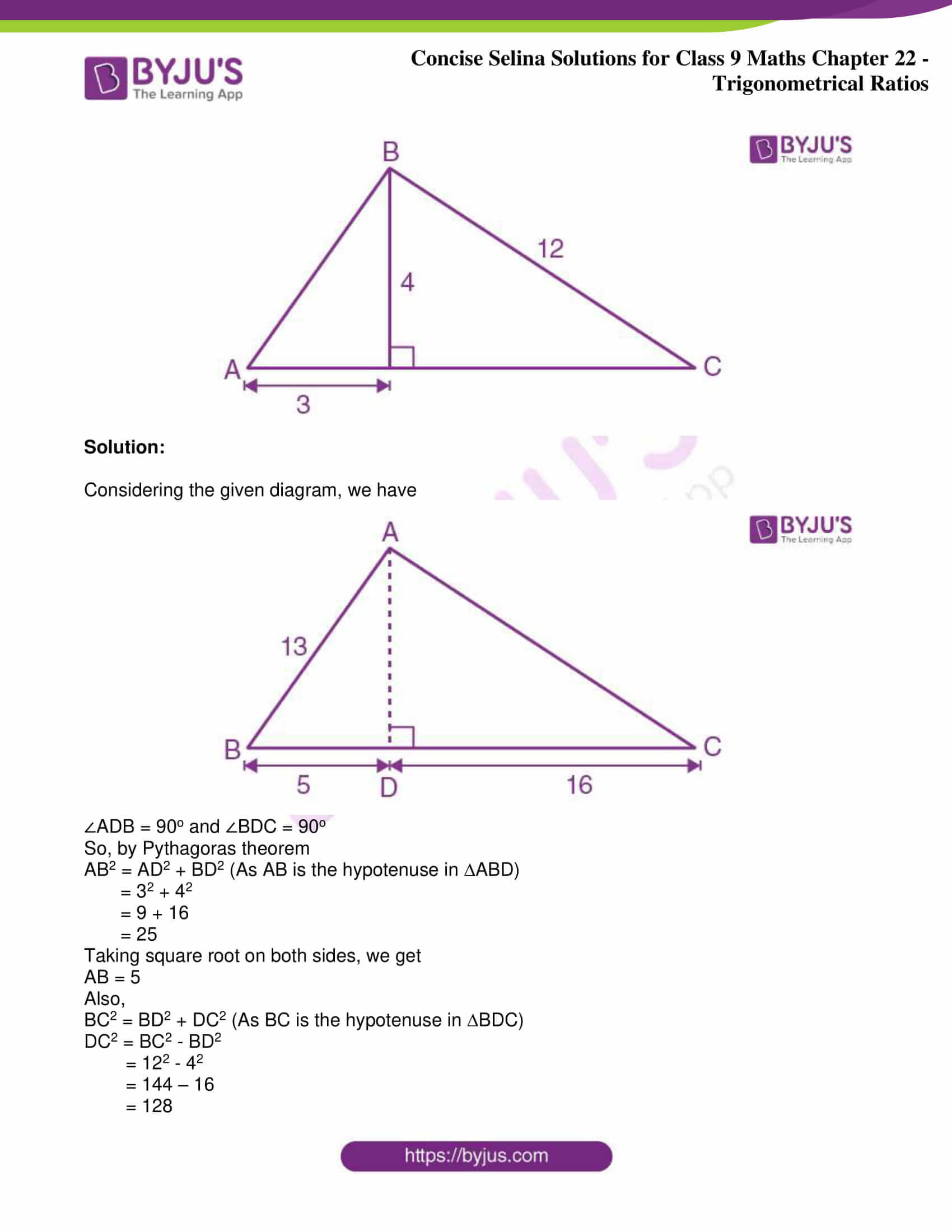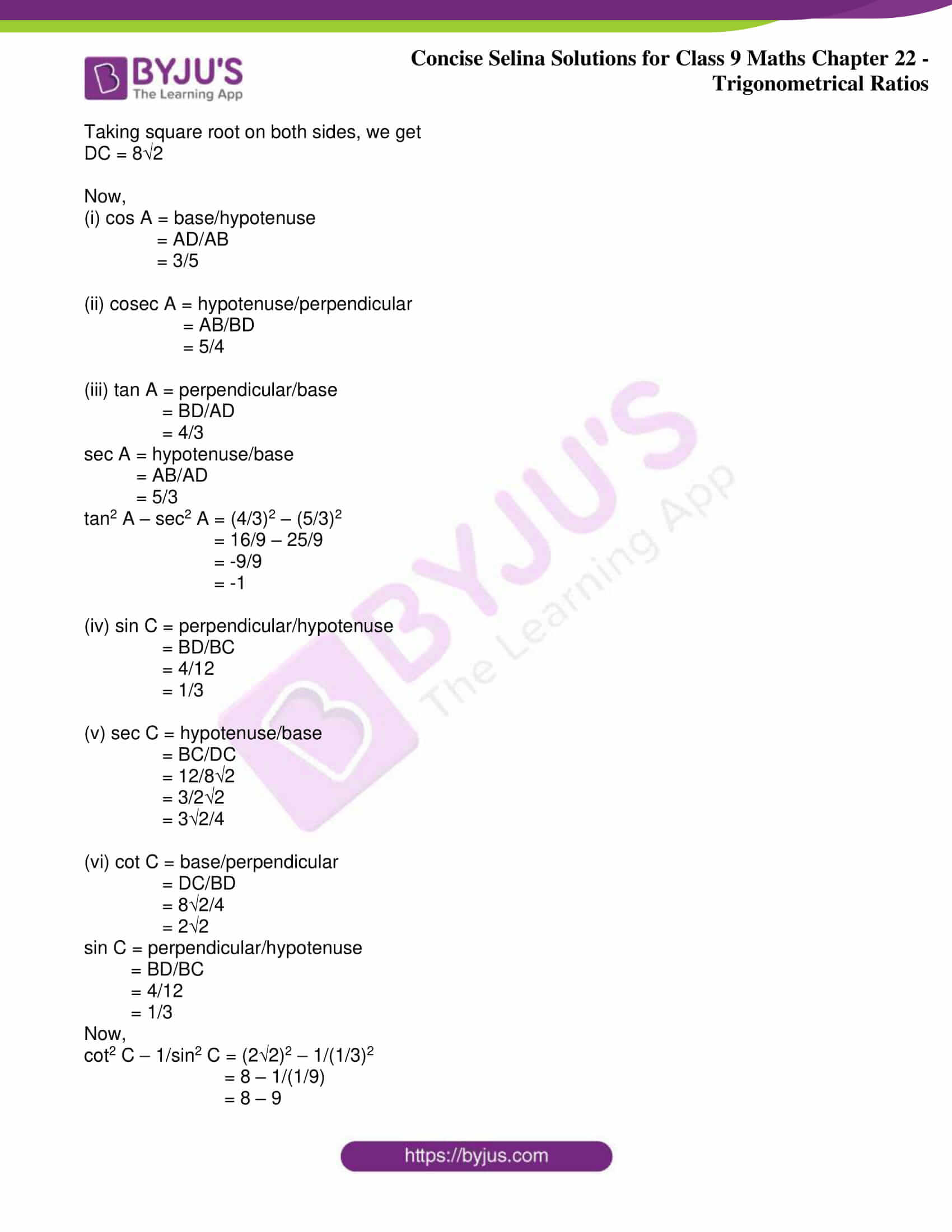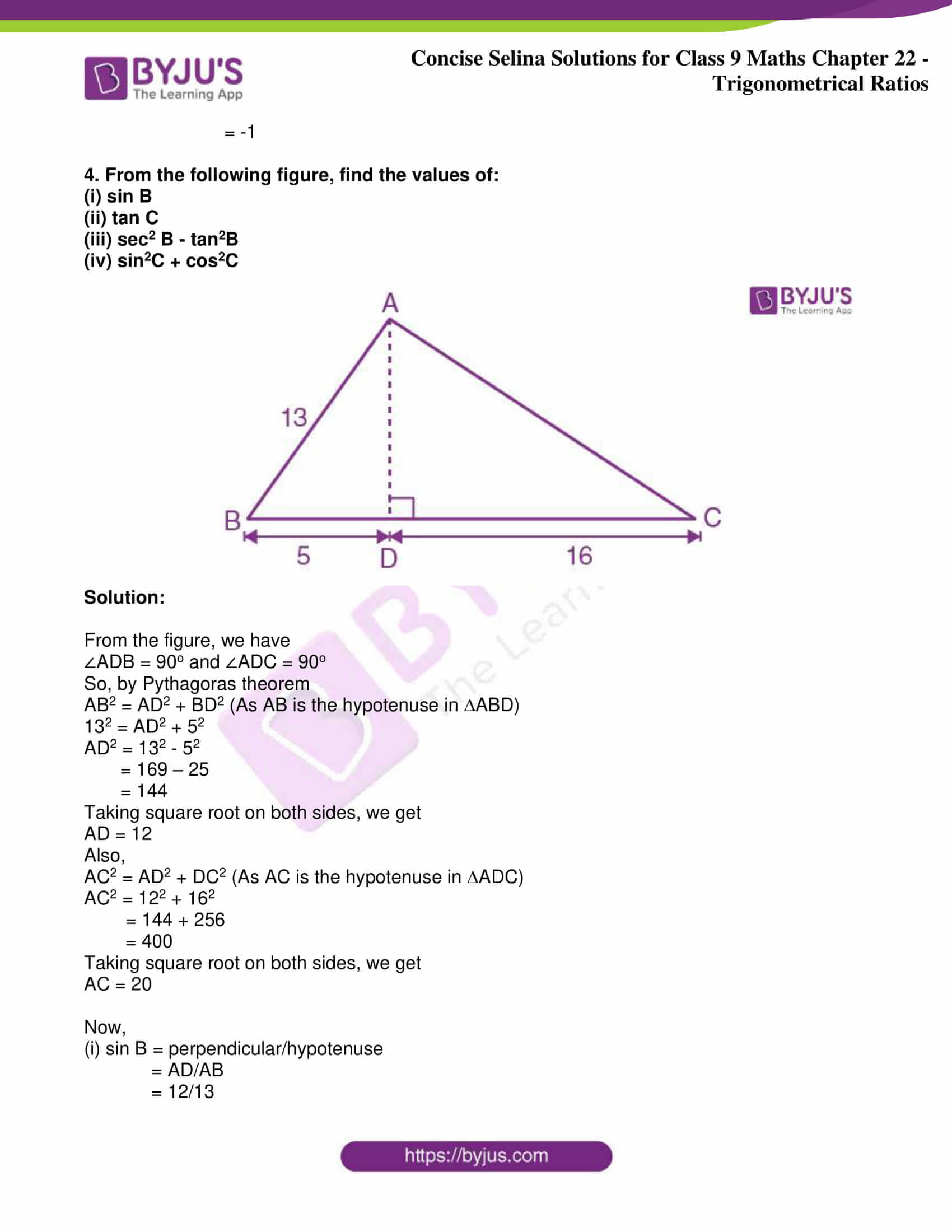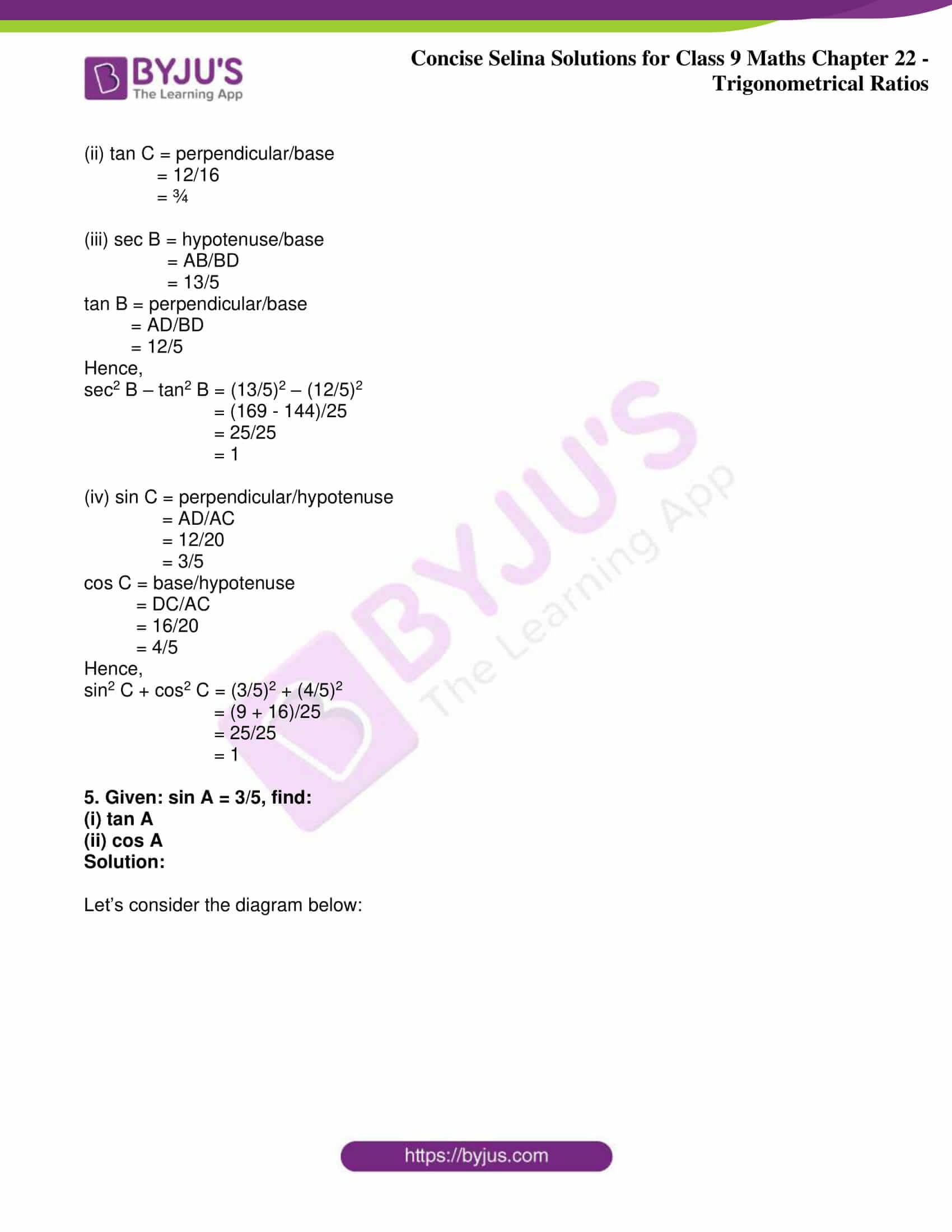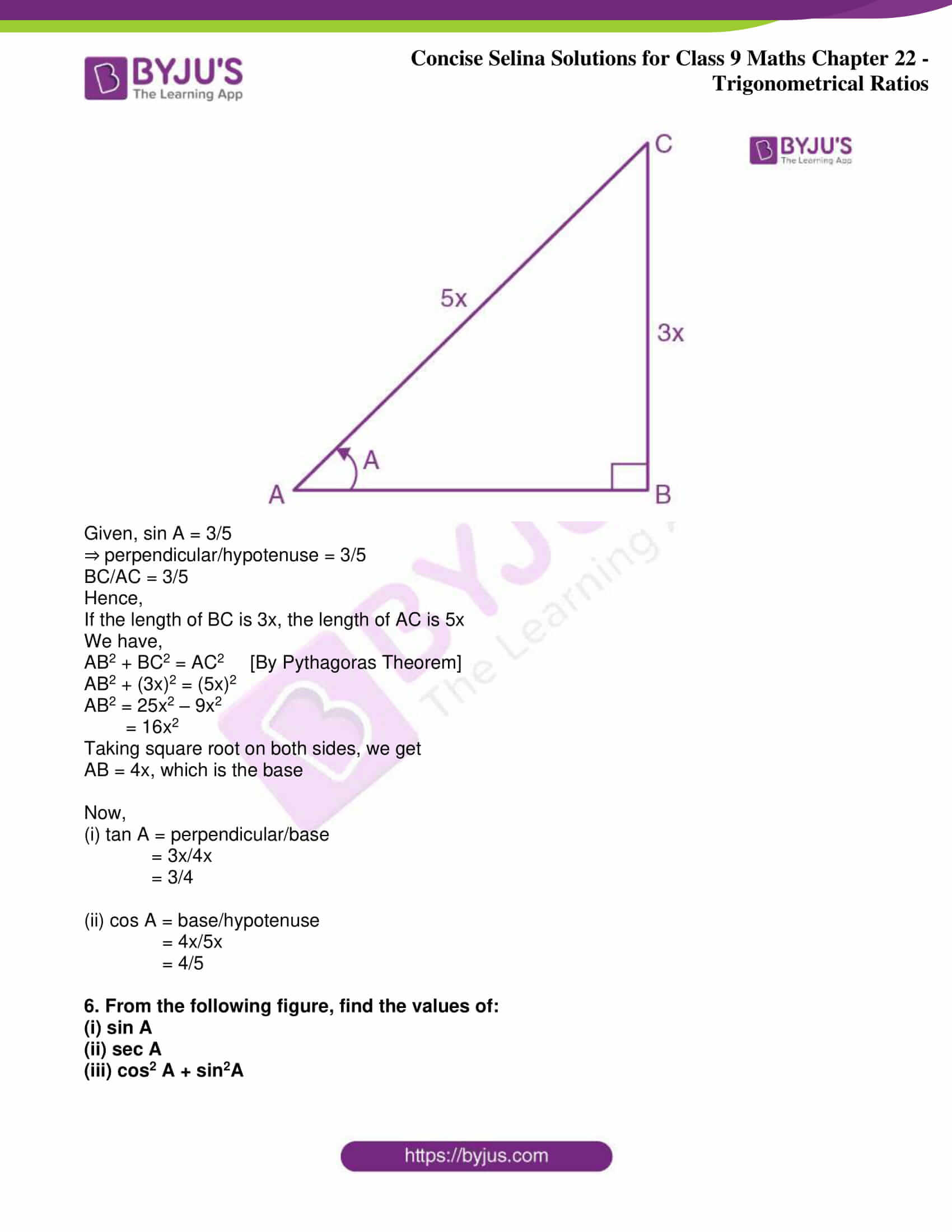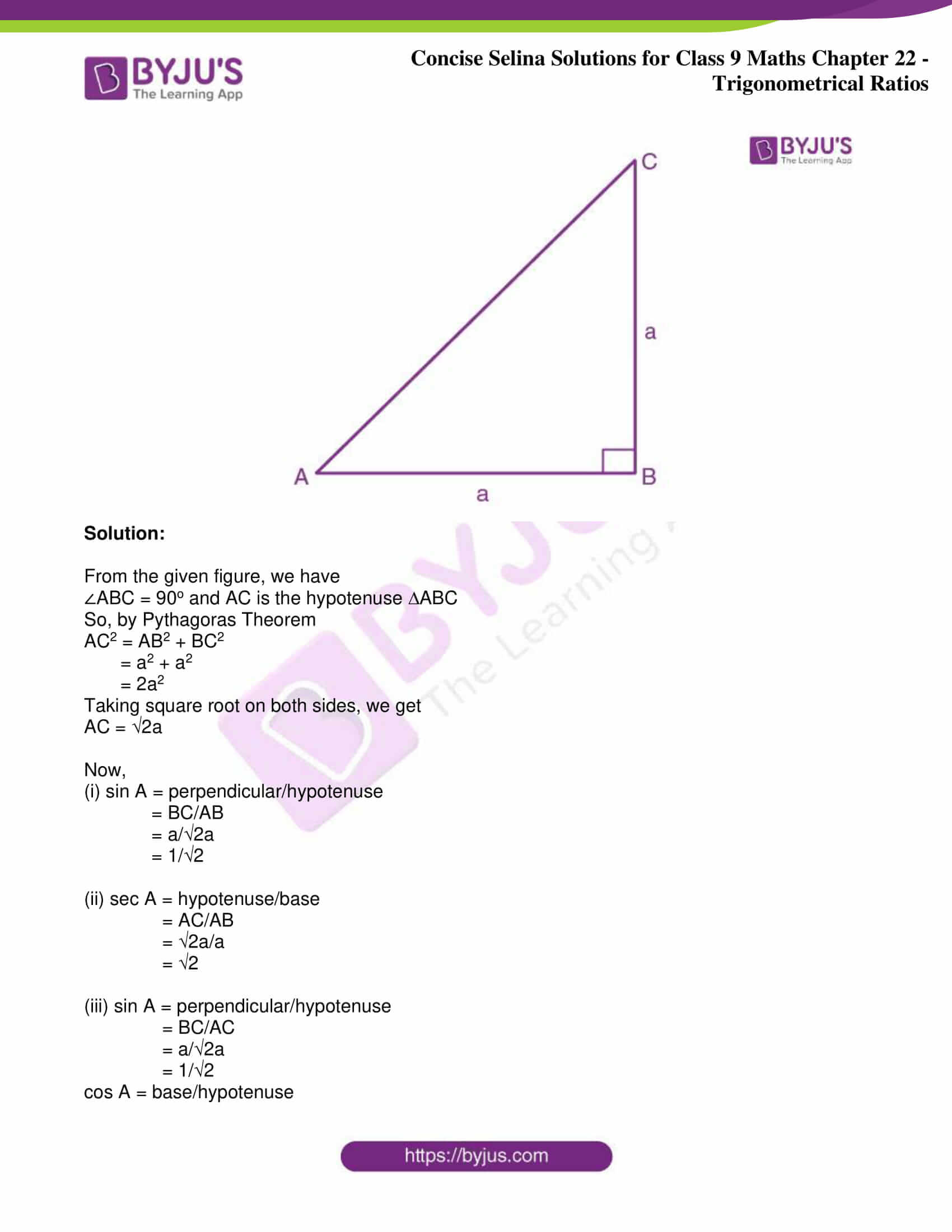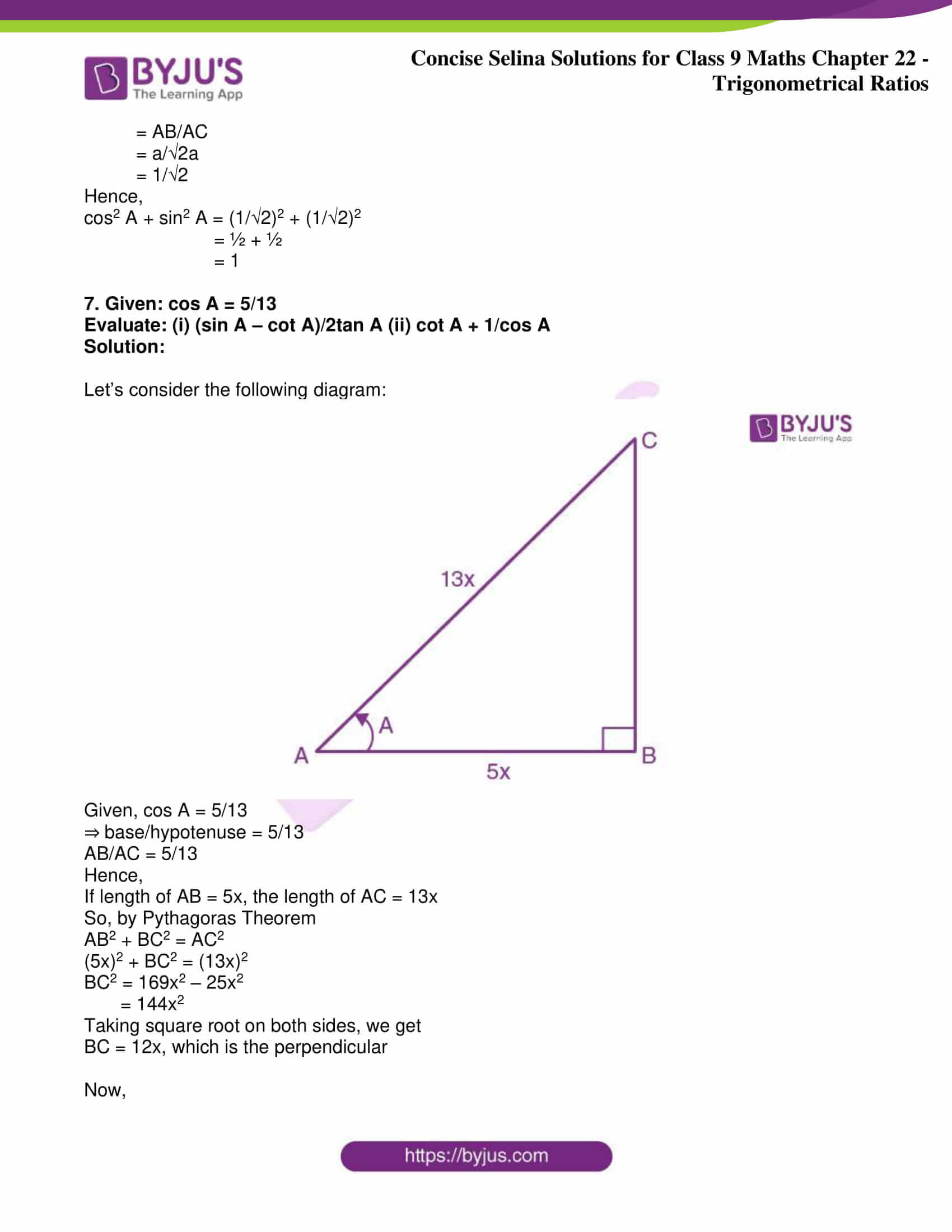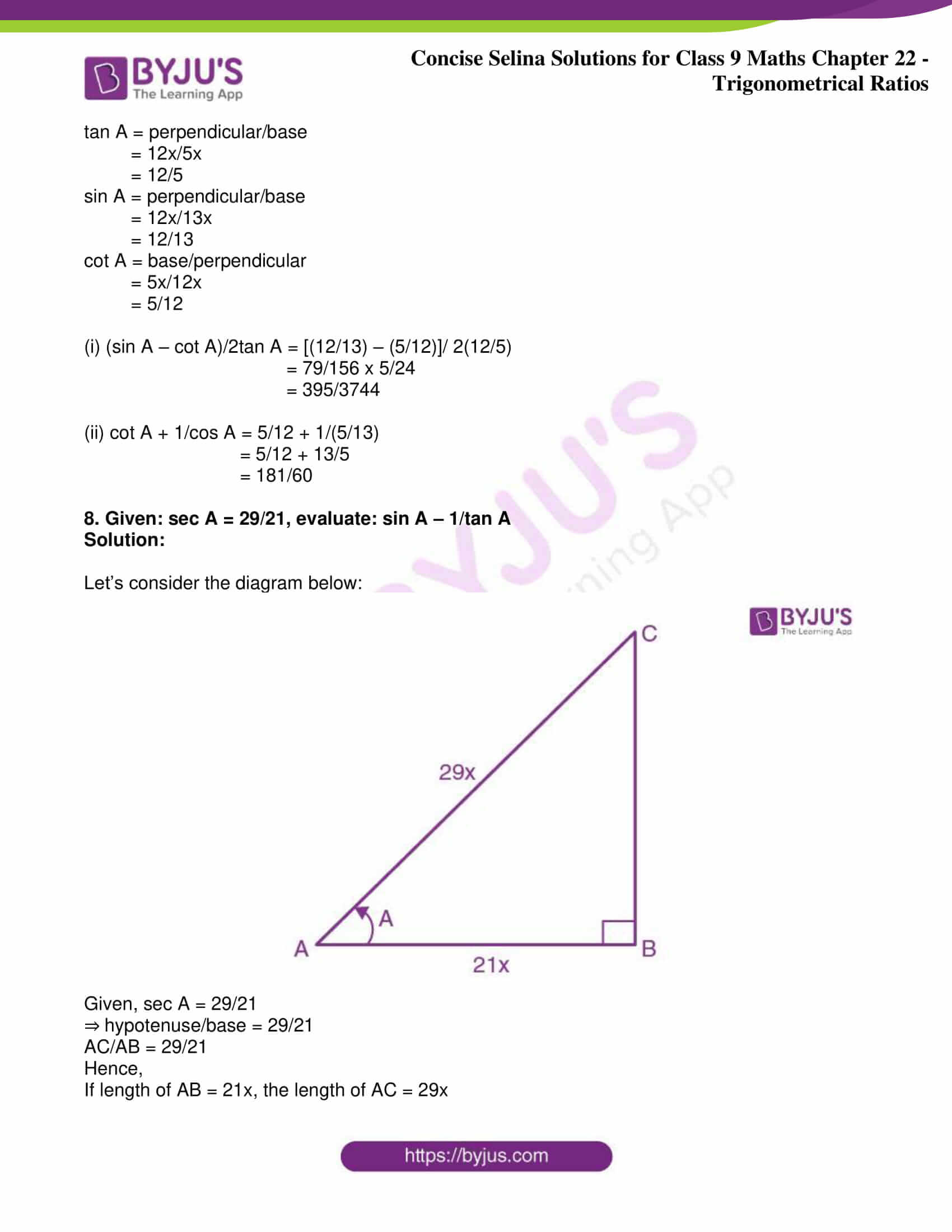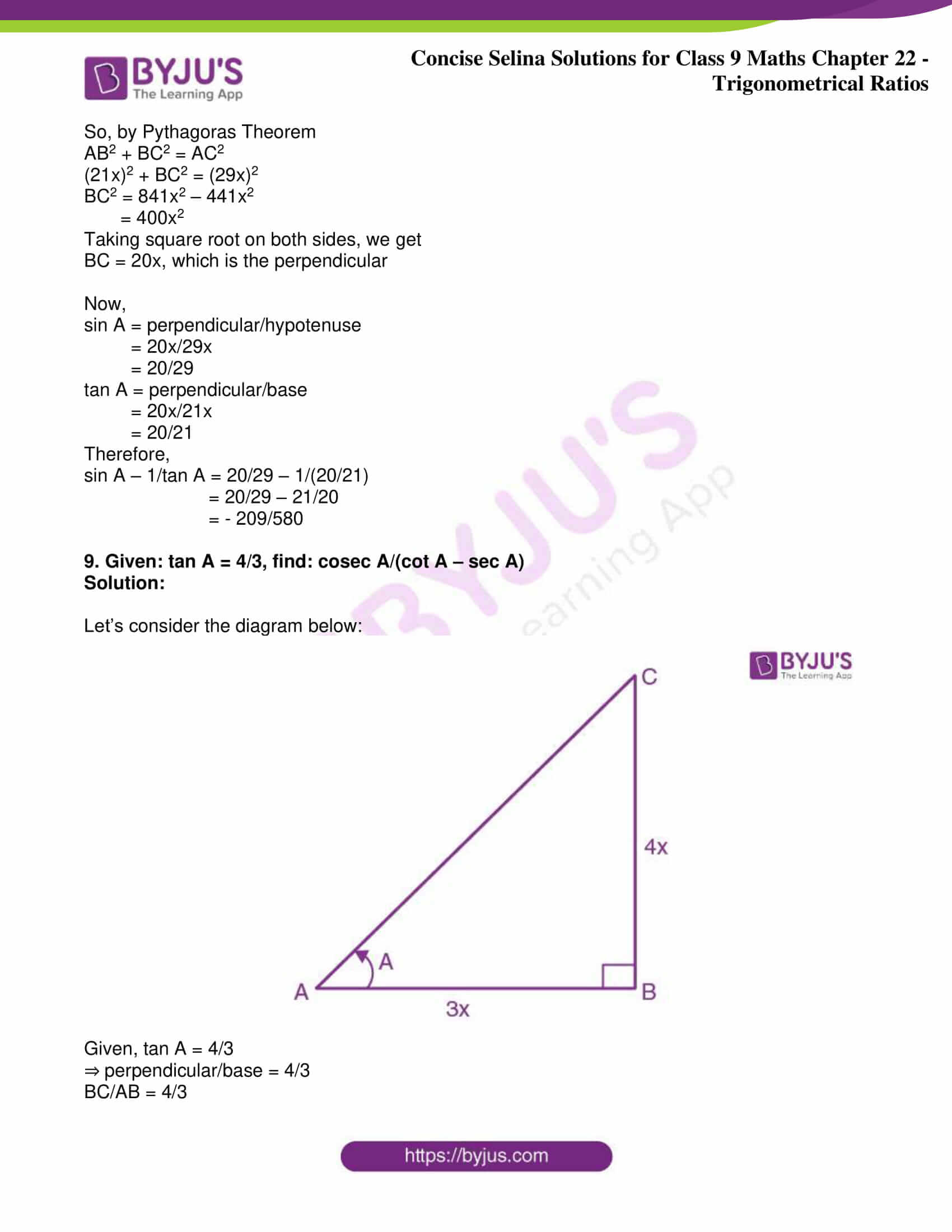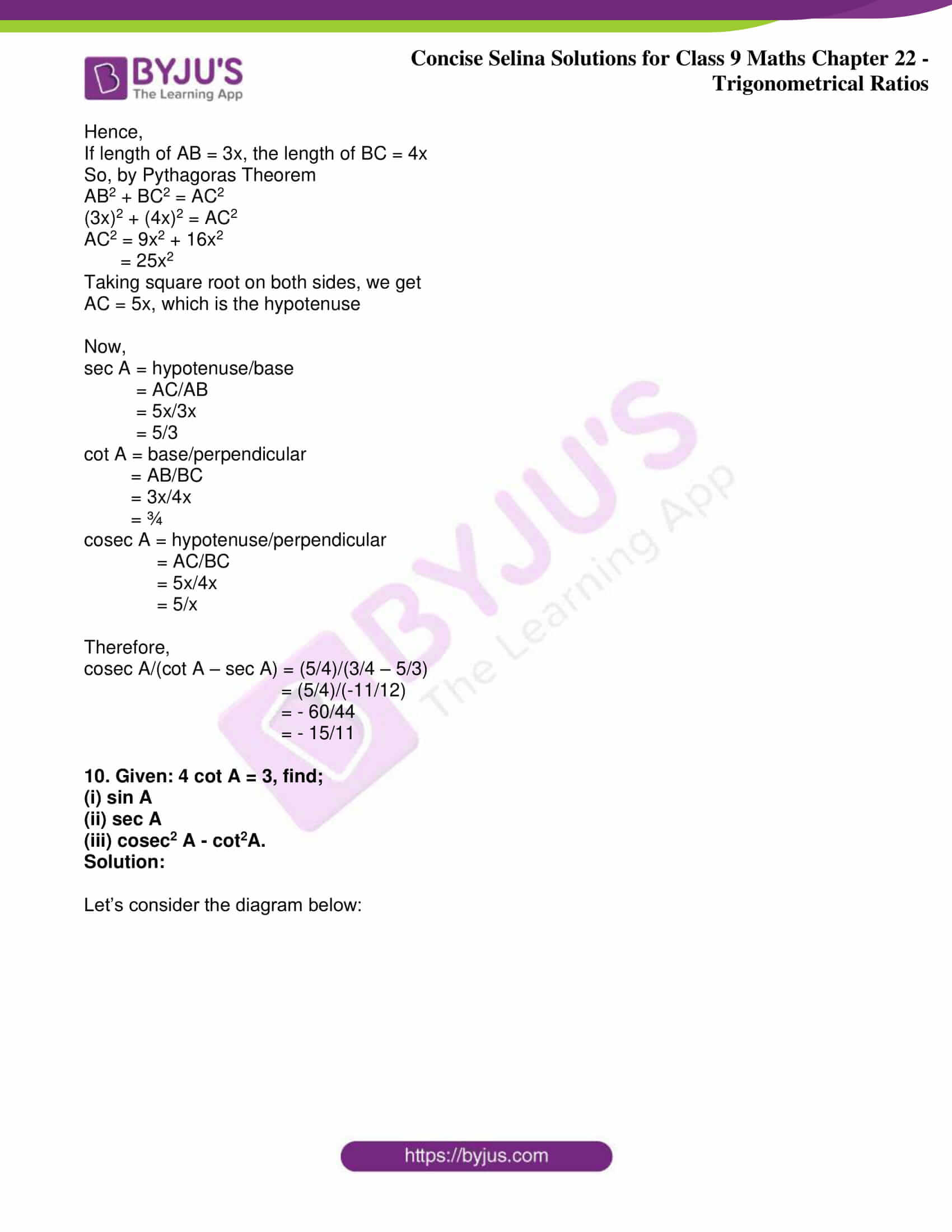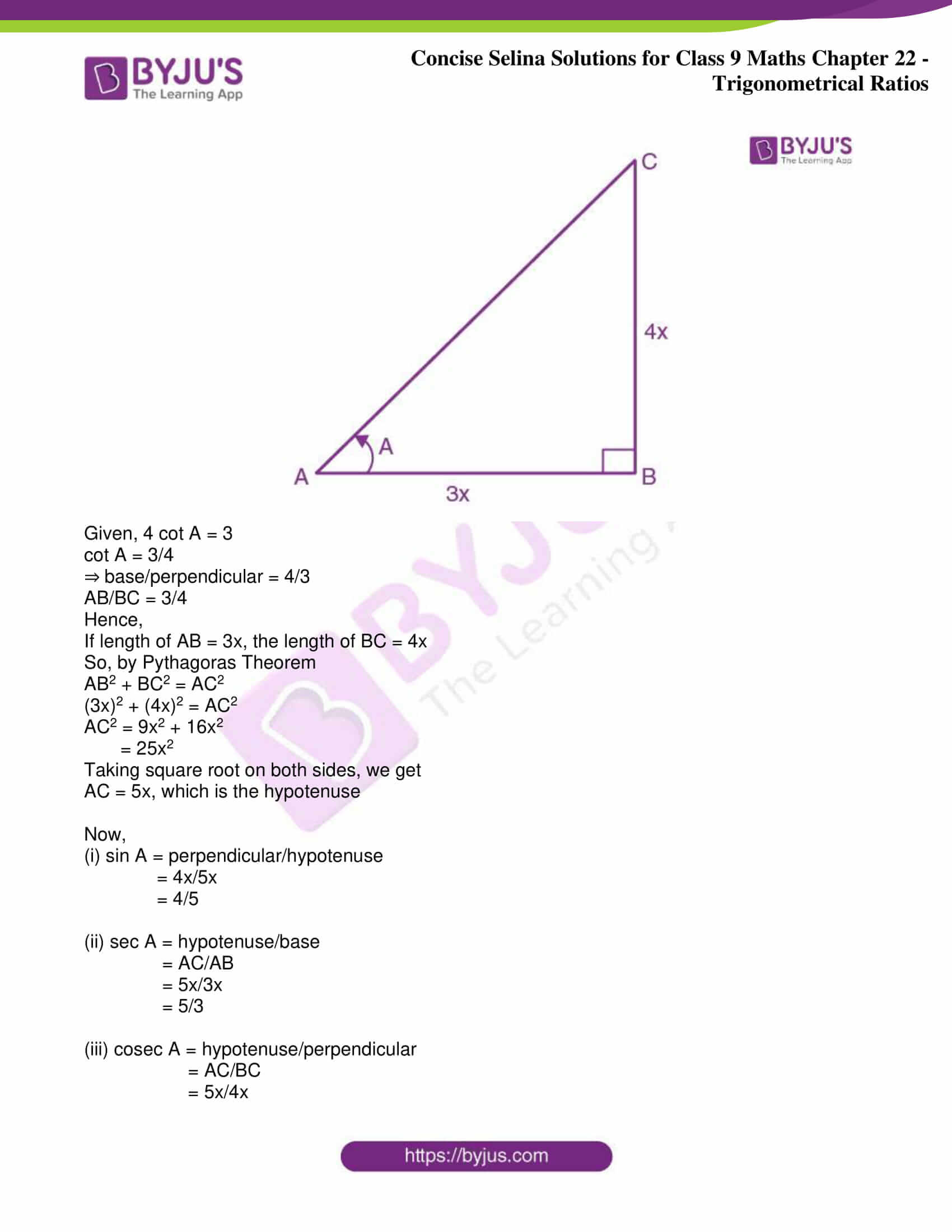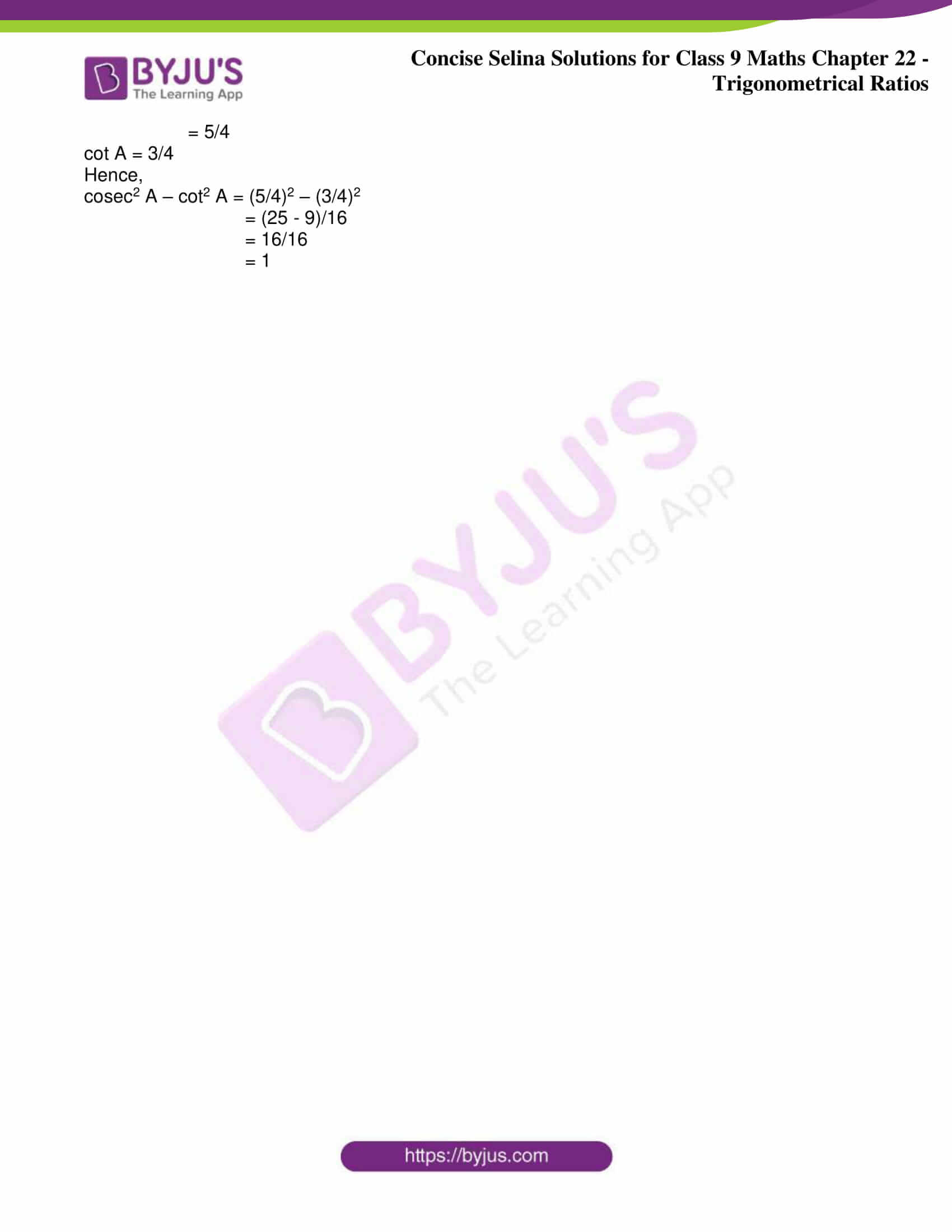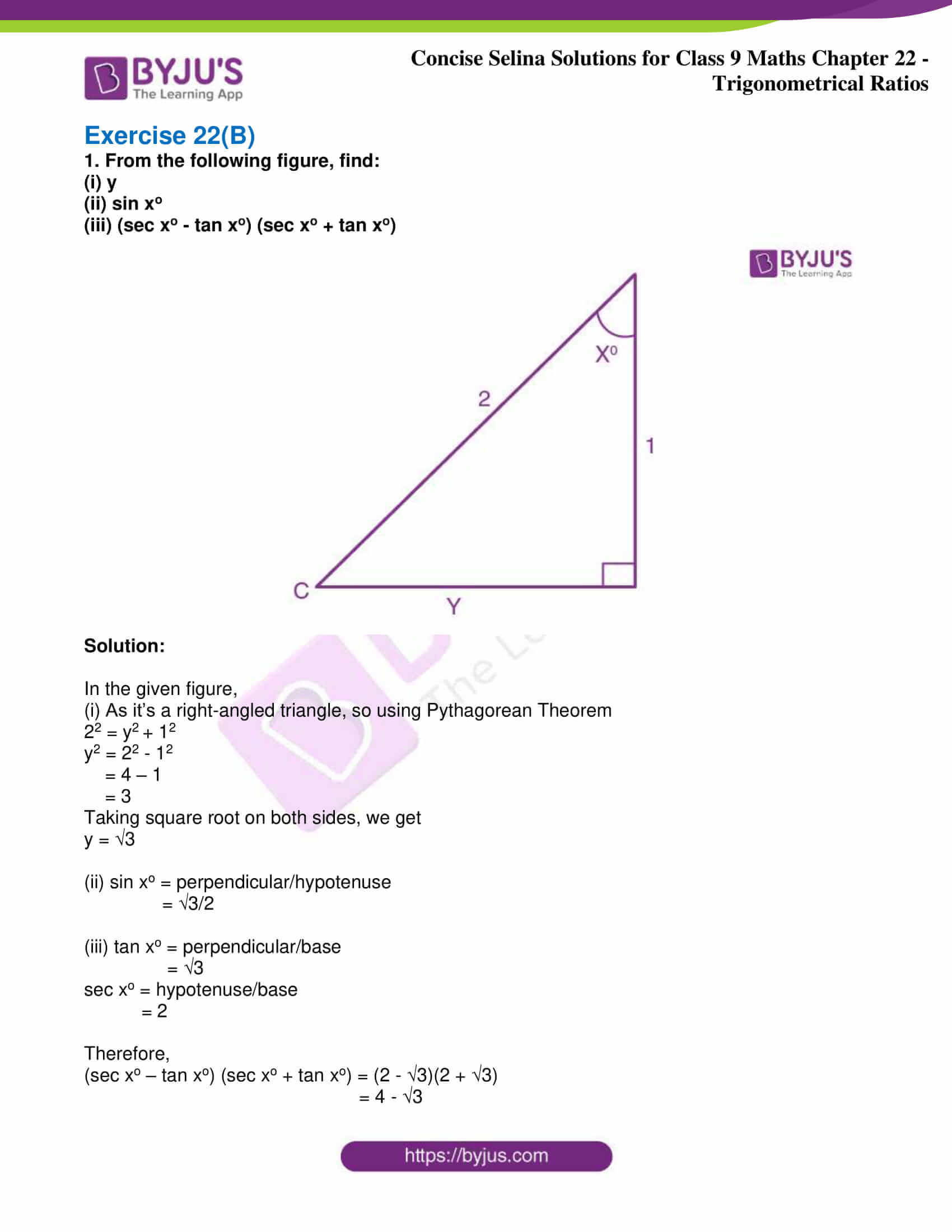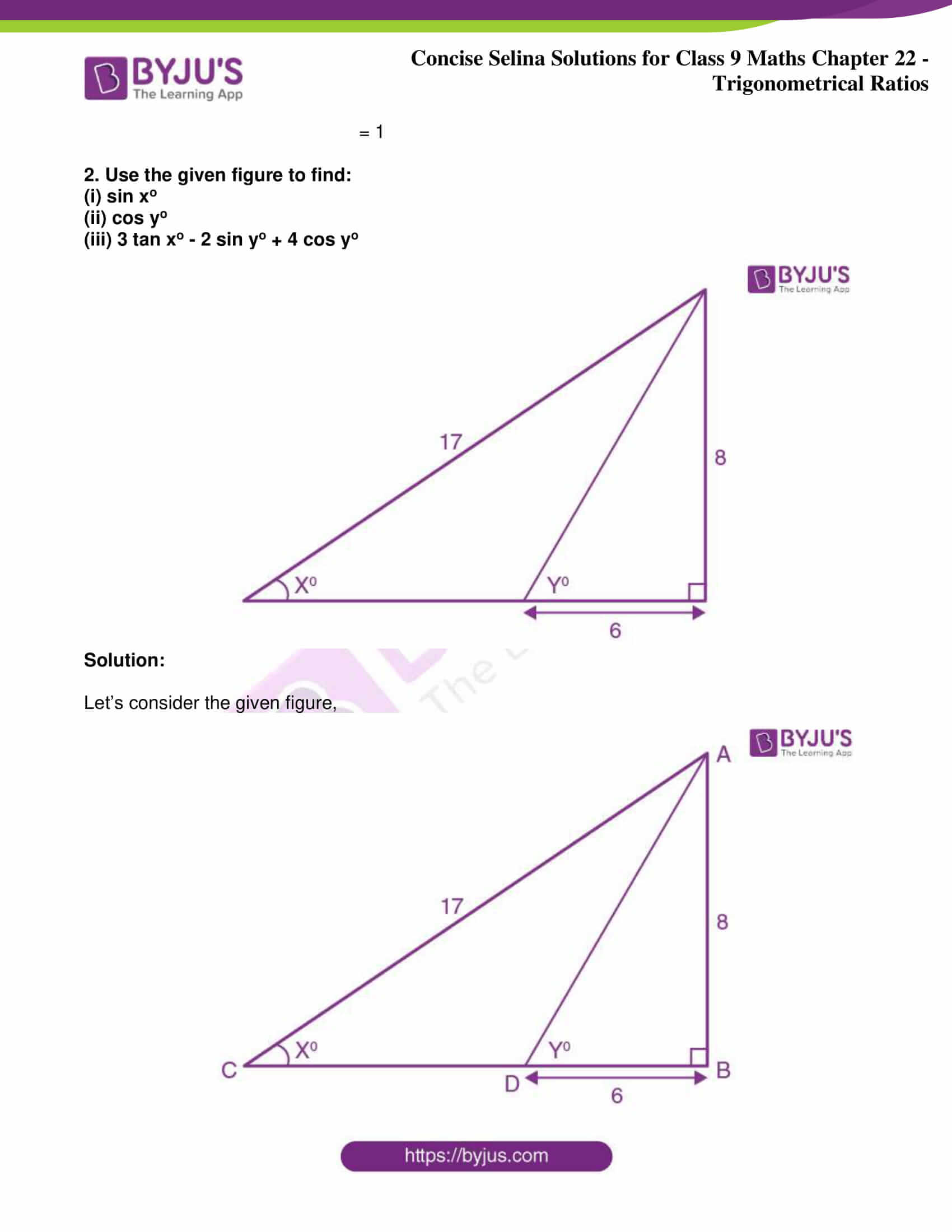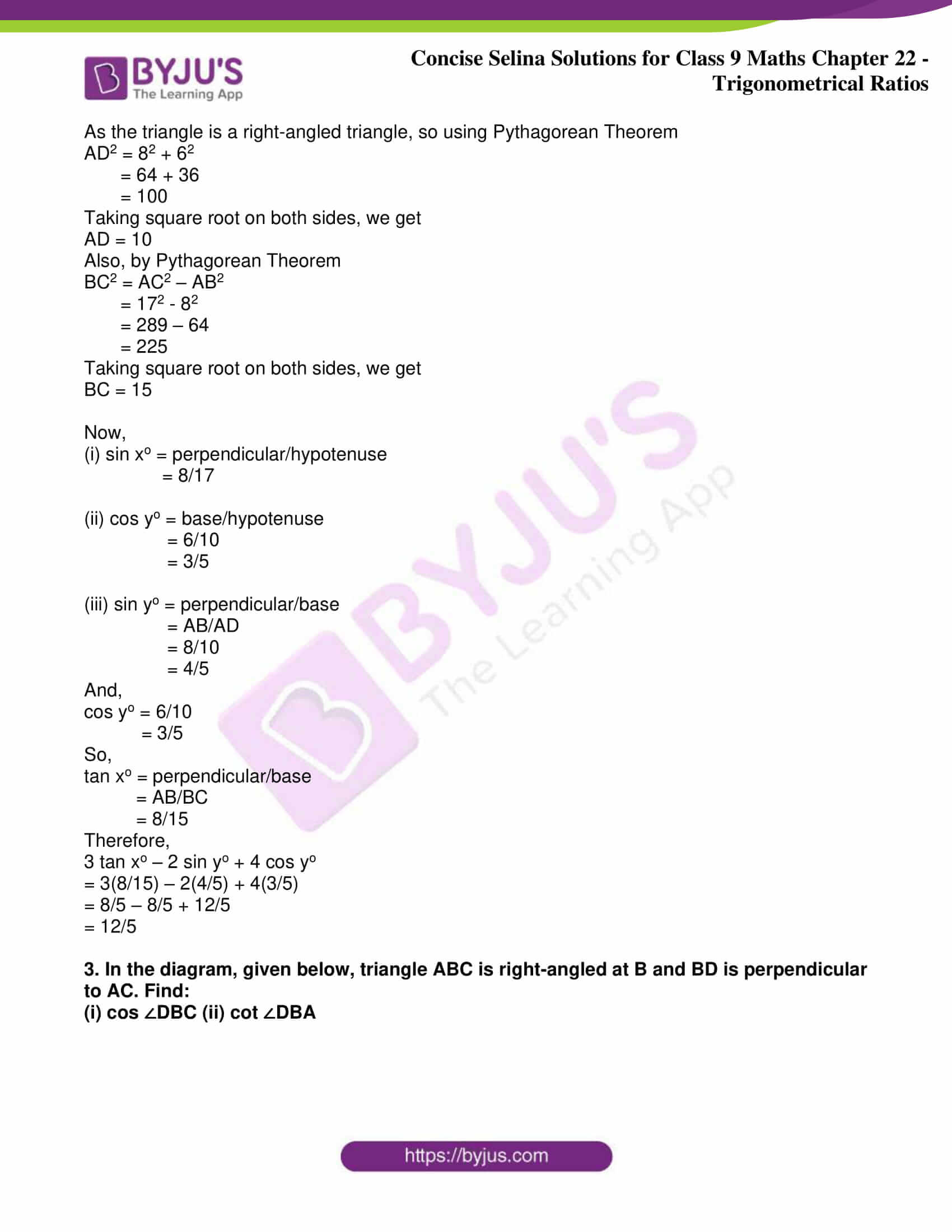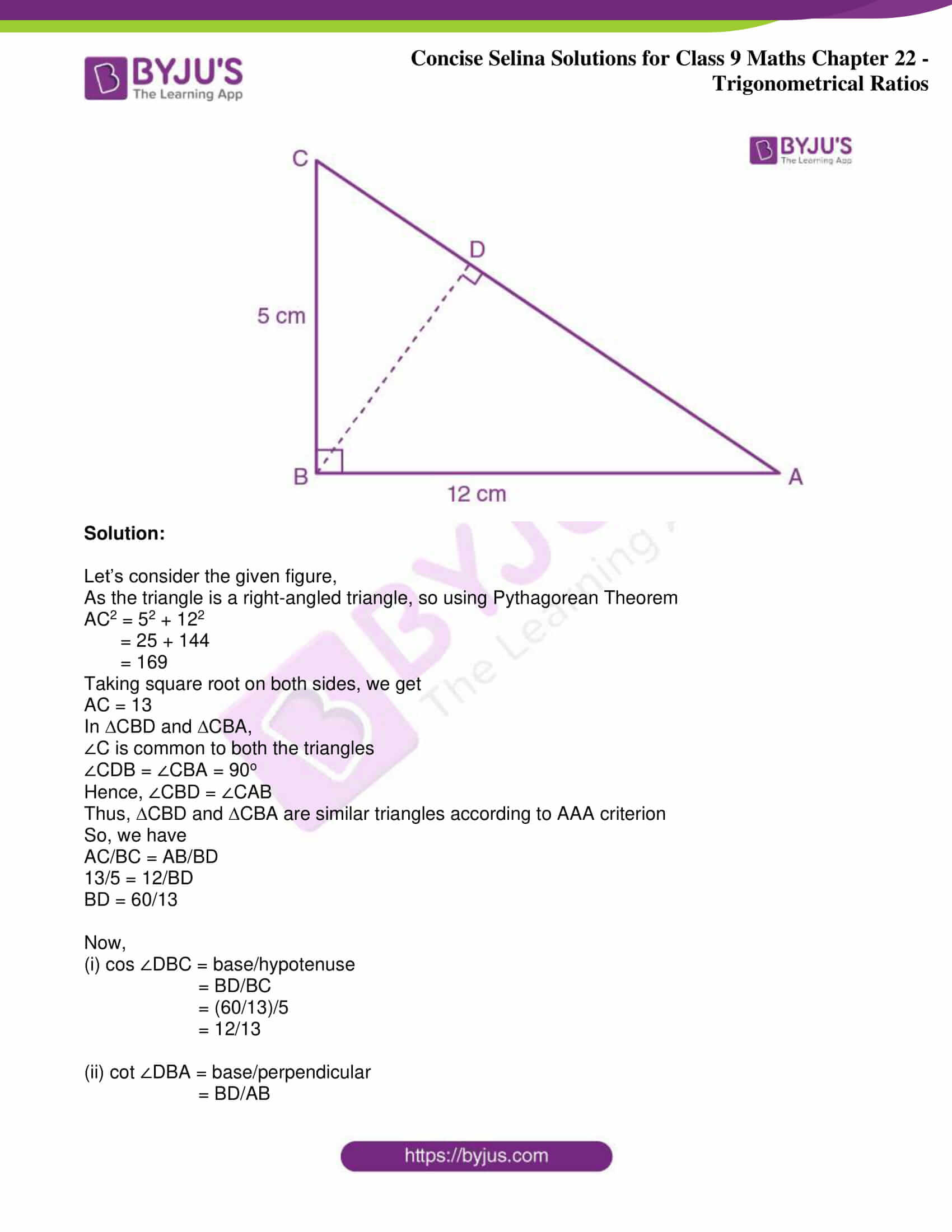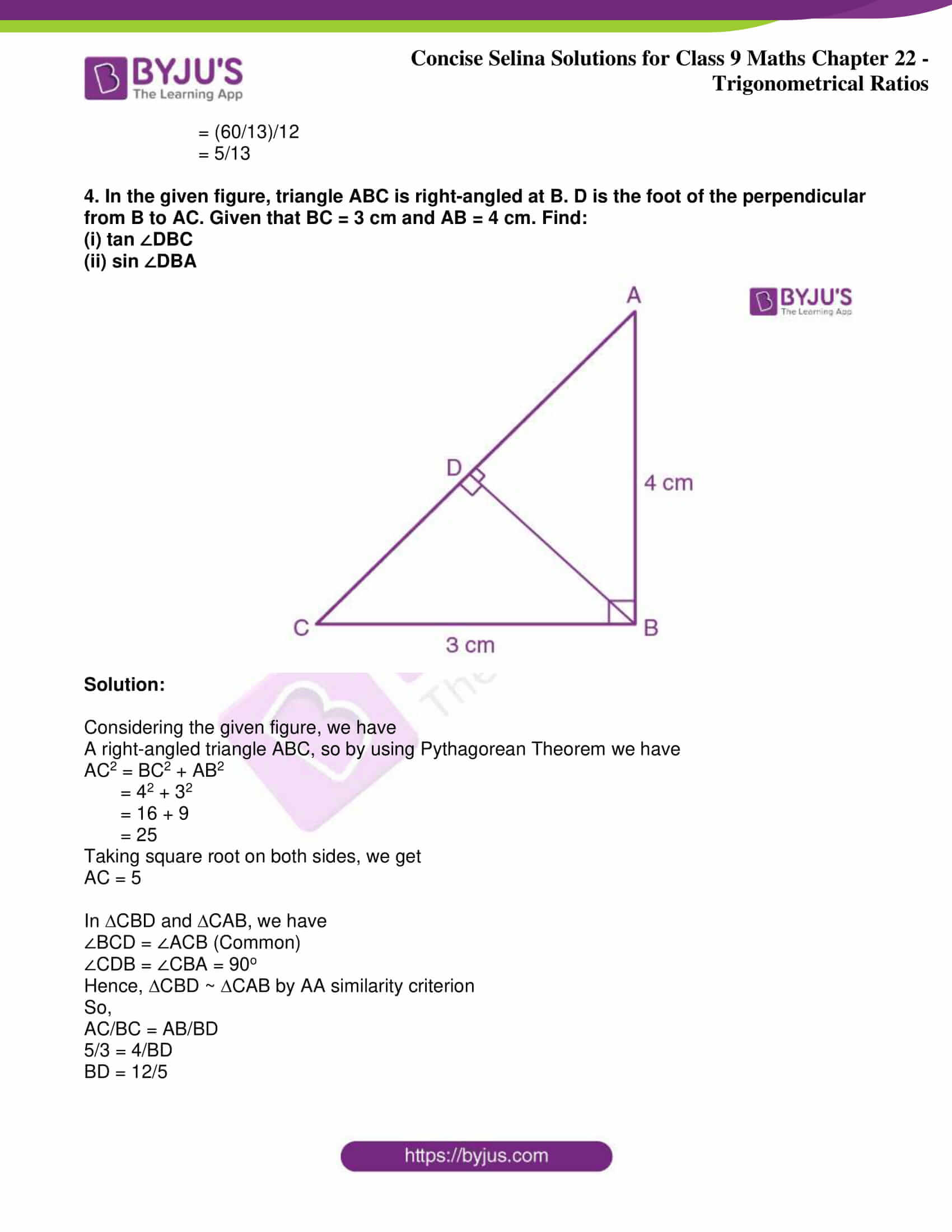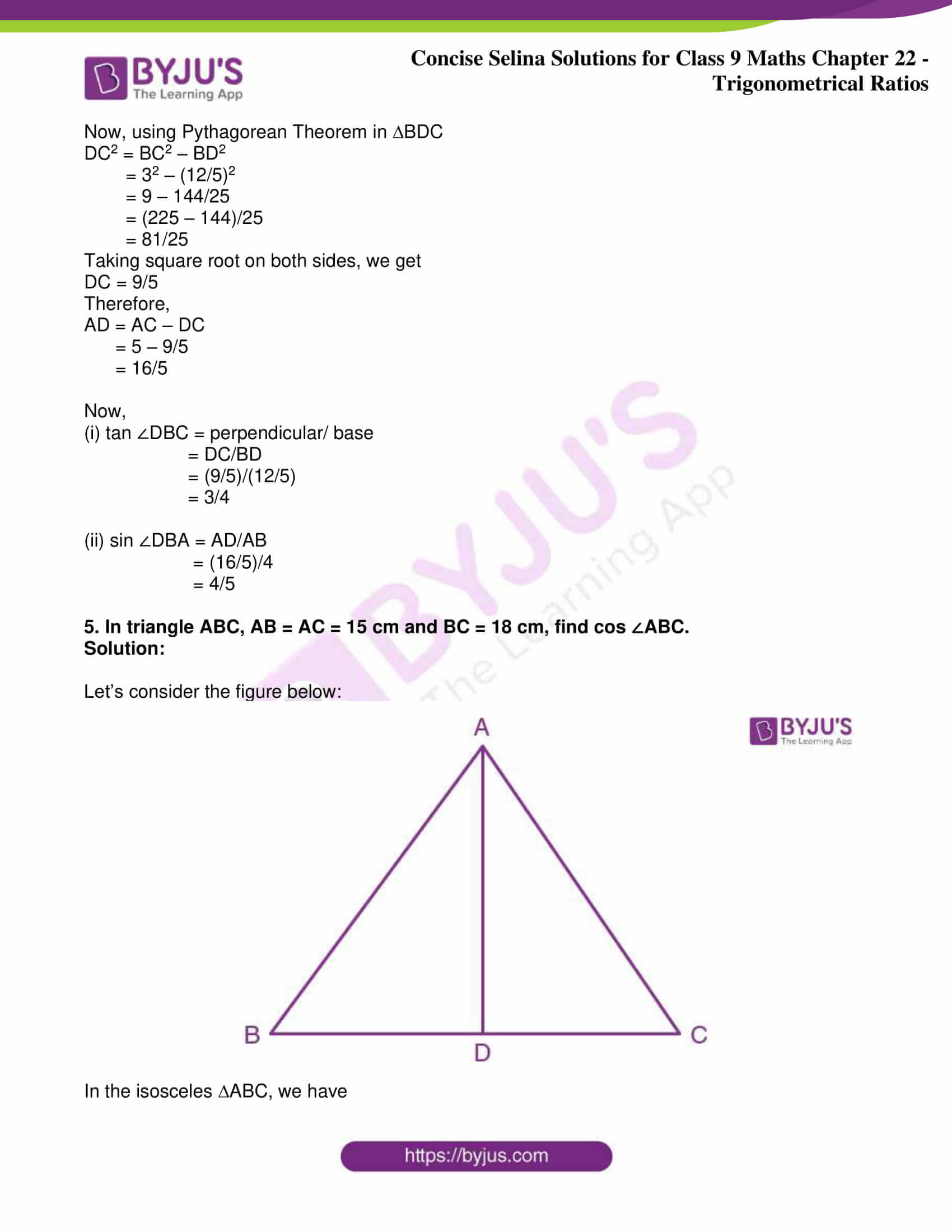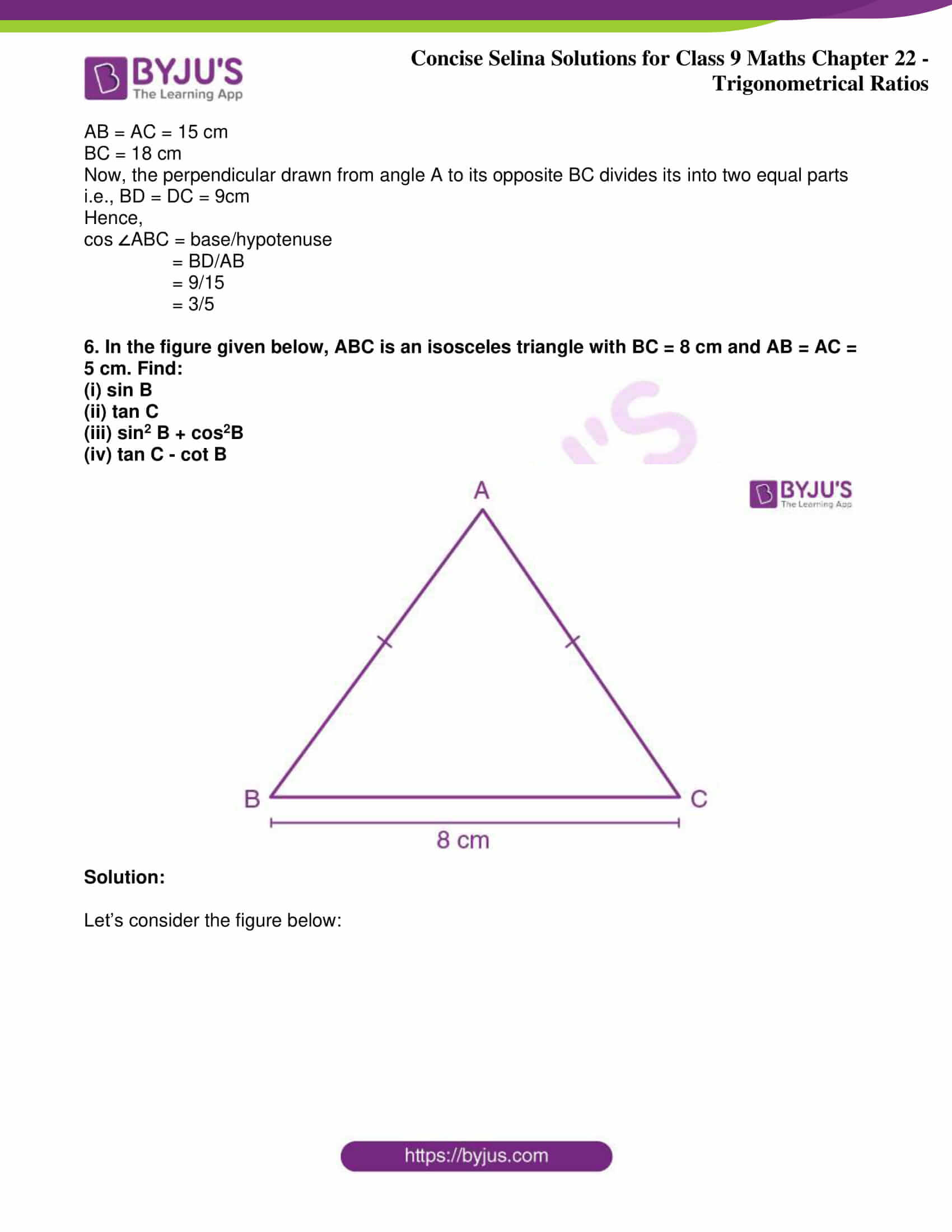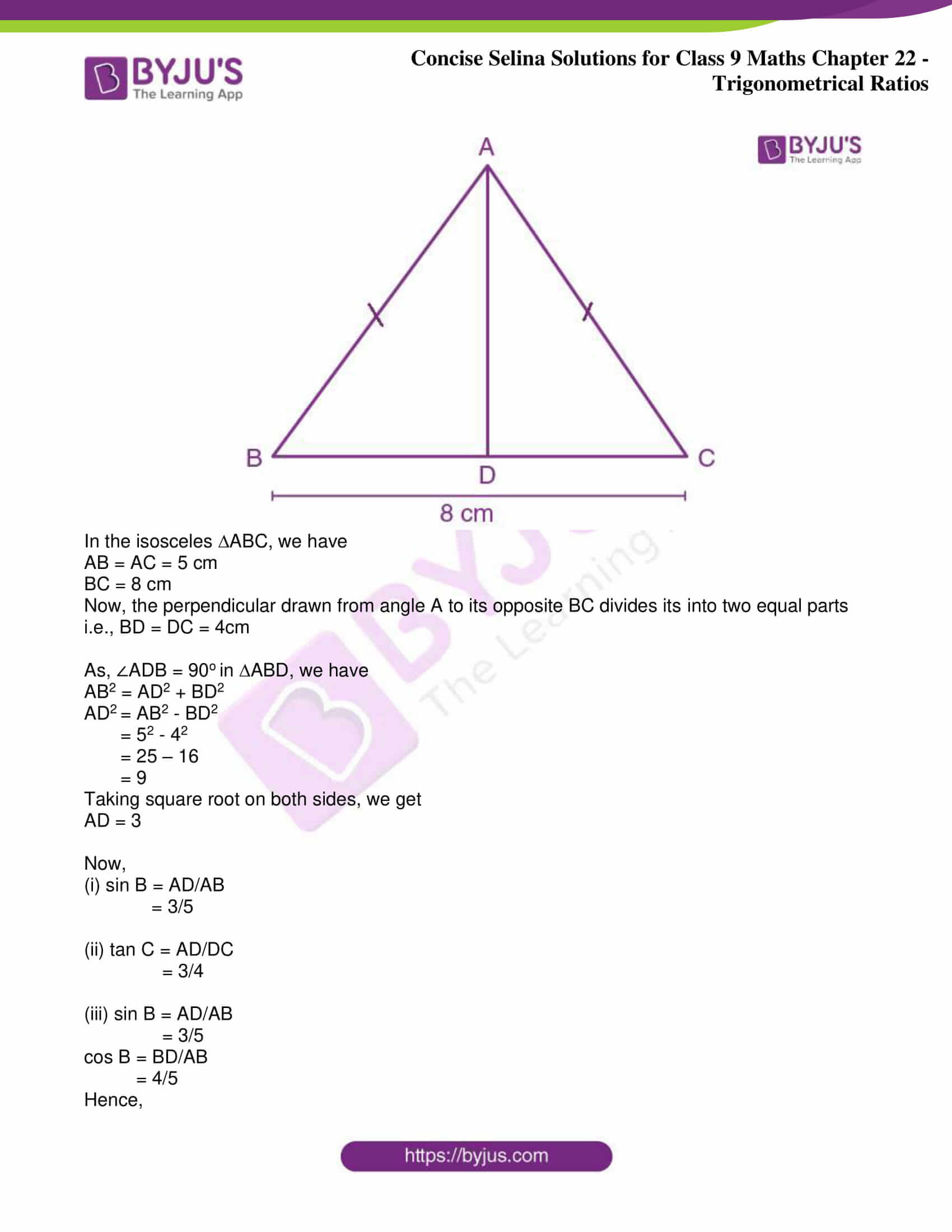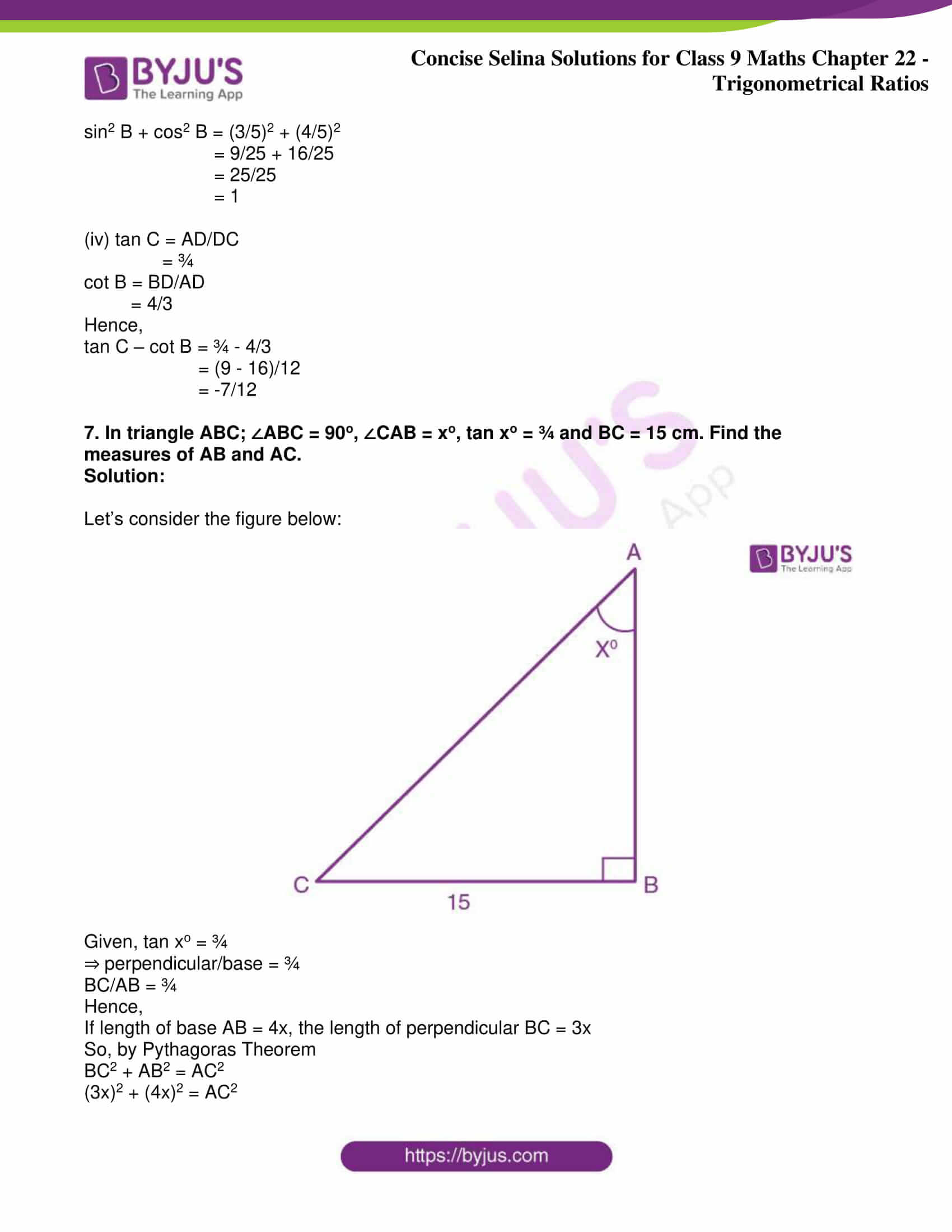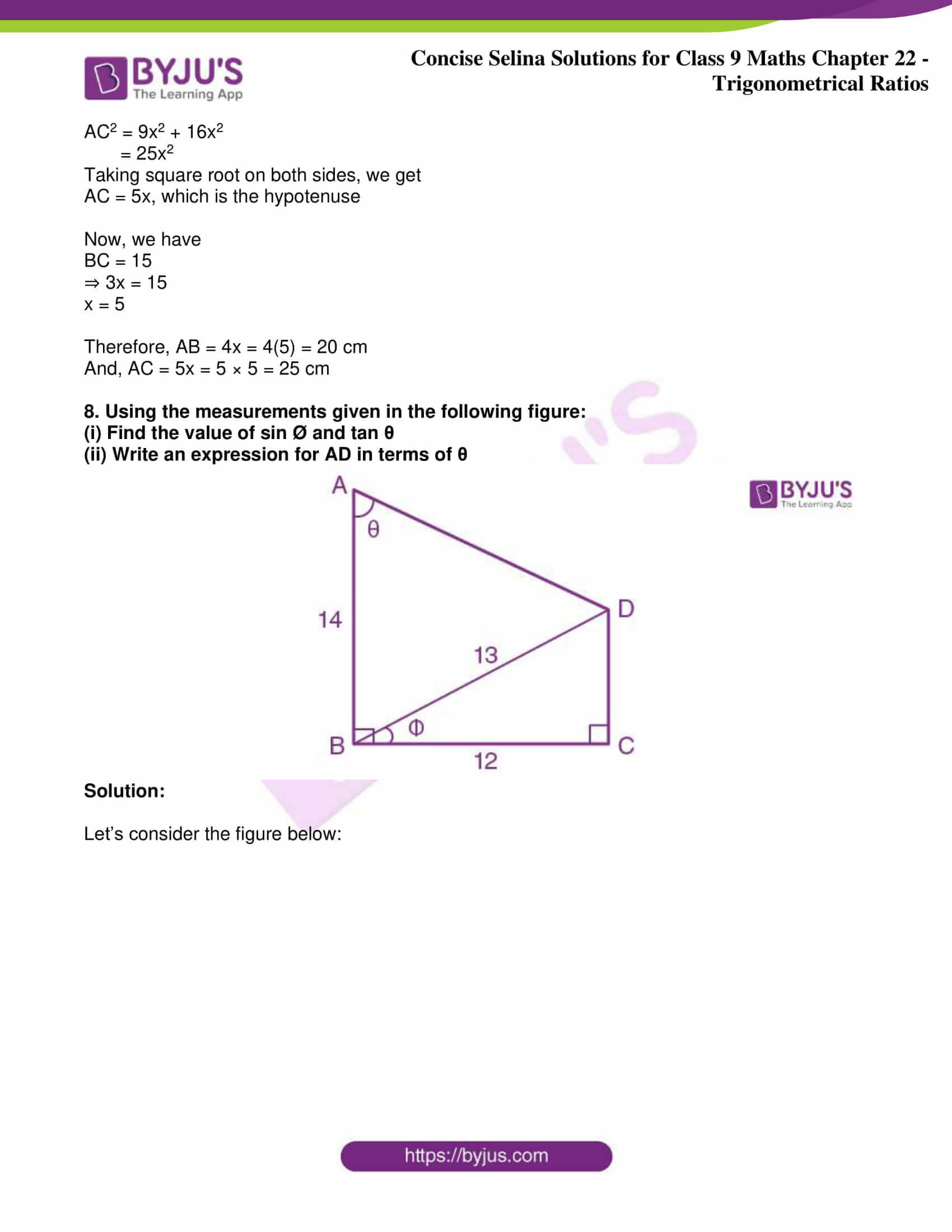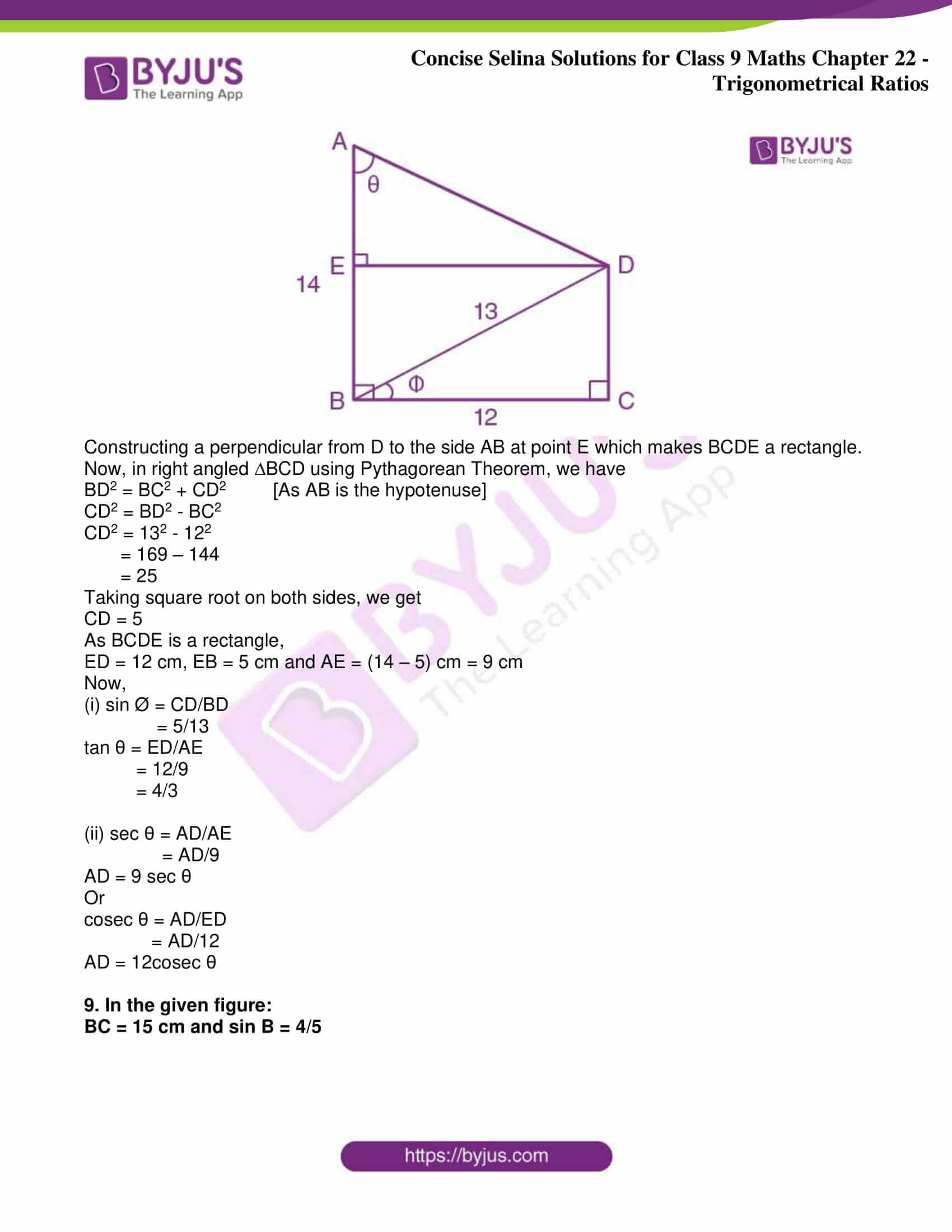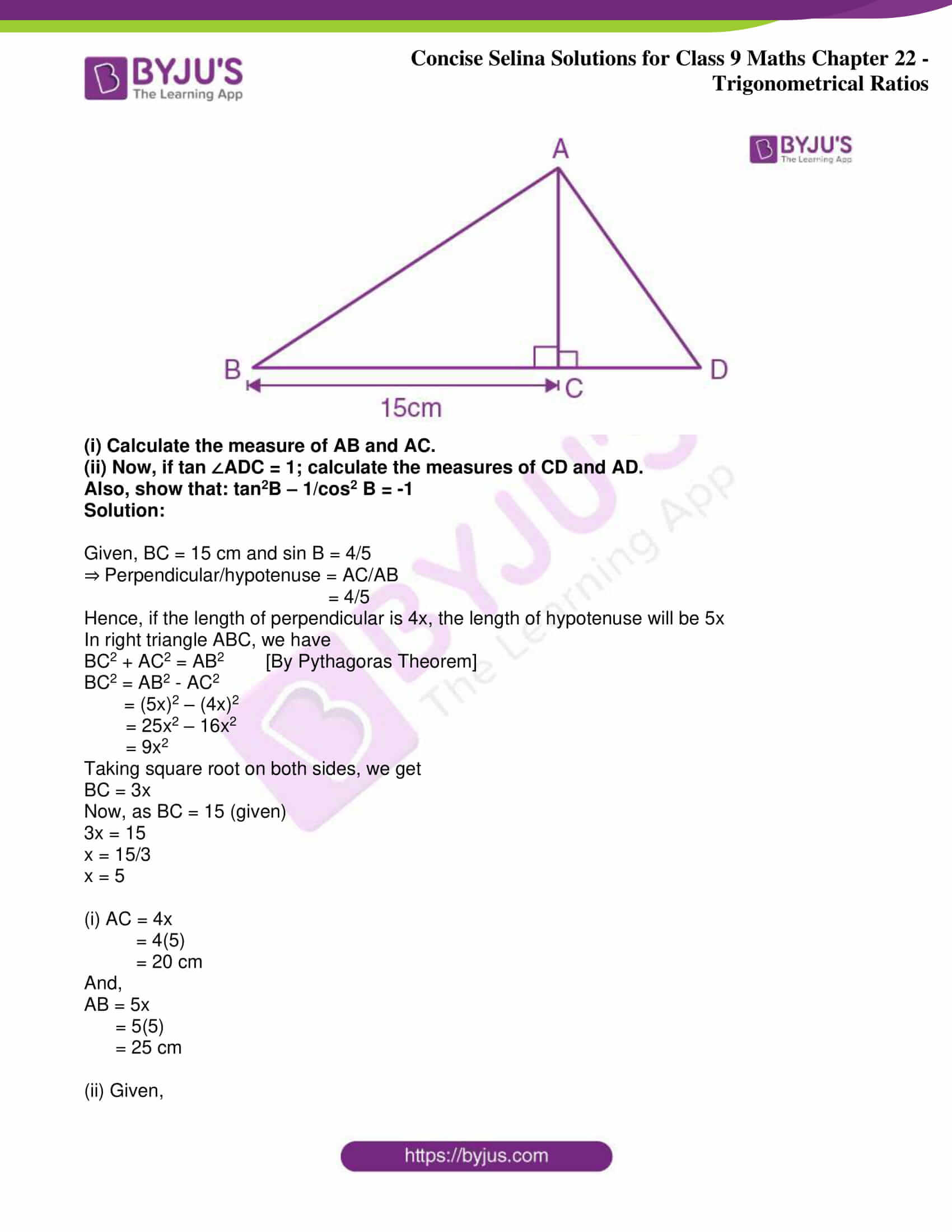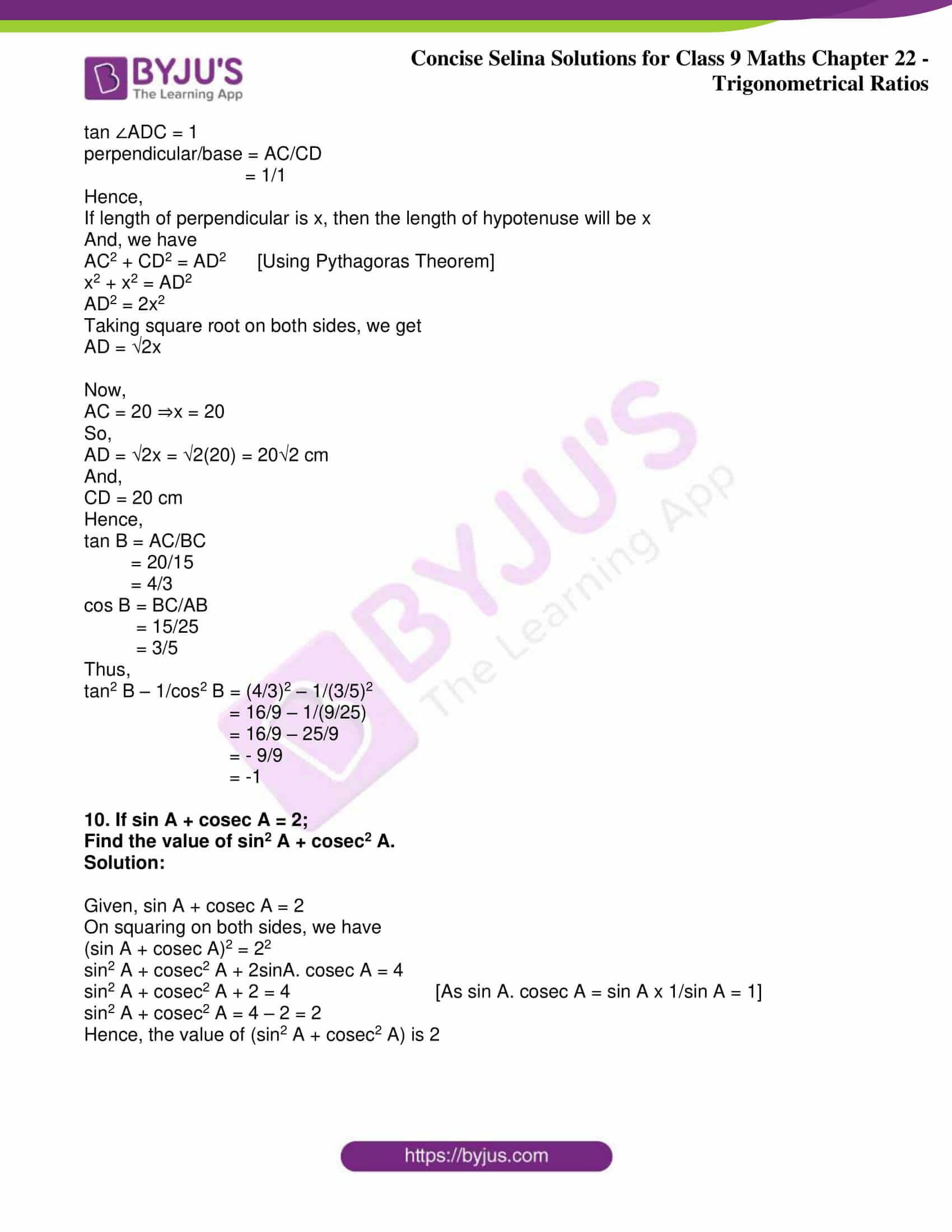Exercise 22(A)

1. From the following figure, find the values of:

(i) sin A

(ii) cos A

(iii) cot A

(iv) sec C

(v) cosec C

(vi) tan C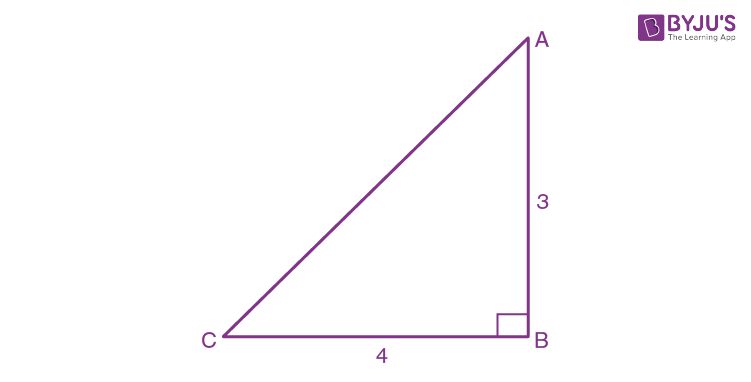Solution:

Given, ∠ABC = 90o

AC2 = AB2 + BC2 (AC is hypotenuse)

AC2 = 32 + 42

= 9 + 16

= 25

Taking square root on both sides, we get

AC = 5 cm

(i) sin A = perpendicular/hypotenuse

= BC/AC

= 4/5

(ii) cos A = base/hypotenuse

= AB/AC

= 3/5

(iii) cot A = base/perpendicular

= AB/BC

= 3/4

(iv) sec C = hypotenuse/base

= AC/BC

= 5/4

(v) cosec C = hypotenuse/perpendicular

= AC/AB

= 5/3

(vi) tan C = perpendicular/base

= AB/BC

= 3/4

2. Form the following figure, find the values of:

(i) cos B

(ii) tan C

(iii) sin2B + cos2B

(iv) sin B. cos C + cos B. sin C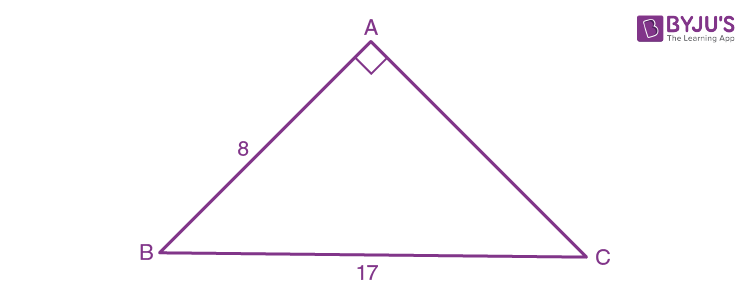Solution:

Given, ∠BAC = 90o

BC2 = AB2 + AC2 (As BC is the hypotenuse)

172 = 82 + AC2

AC2 = 289 – 64

= 225

Taking square root on both sides, we get

AC = 15 cm

(i) cos B = base/hypotenuse

= AB/BC

= 8/17

(ii) tan C = perpendicular/base

= AB/AC

= 8/15

(iii) sin B = perpendicular/hypotenuse

= AC/BC

= 15/17

cos B = base/hypotenuse

= AB/BC

= 8/17

Now,

sin2 B + cos2 B = (15/17)2 + (8/17)2

= (225 + 64)/289

= 289/289

= 1

(iv) sin B = perpendicular/hypotenuse

= AC/BC

= 15/17

cos B = base/hypotenuse

= AB/BC

= 8/17

sin C = perpendicular/hypotenuse

= AB/BC

= 8/17

cos C = base/hypotenuse

= AC/BC

= 15/17

Now,

sin B. cos C + cos B. sin C = 15/17 x 15/17 + 8/17 x 8/17

= (225 + 64)/289

= 289/289

= 1

3. From the following figure, find the values of:

(i) cos A

(ii) cosec A

(iii) tan2A – sec2

(iv) sin C

(v) sec C

(vi) cot2 C – 1/sin2 C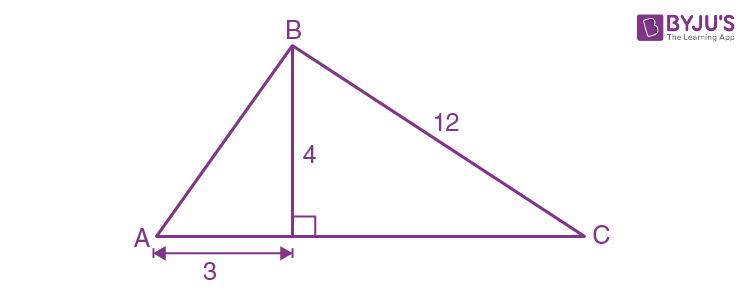Solution:

Considering the given diagram, we have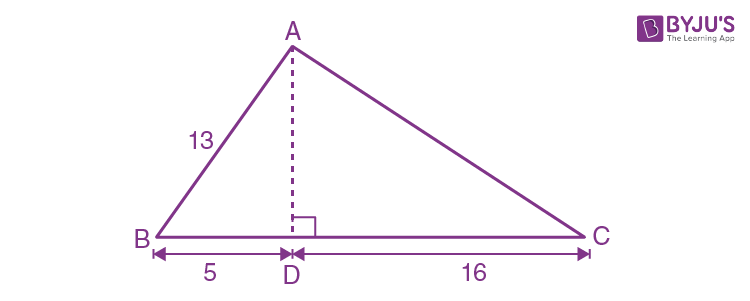∠ADB = 90o and ∠BDC = 90o

So, by Pythagoras theorem

AB2 = AD2 + BD2 (As AB is the hypotenuse in ∆ABD)

= 32 + 42

= 9 + 16

= 25

Taking square root on both sides, we get

AB = 5

Also,

BC2 = BD2 + DC2 (As BC is the hypotenuse in ∆BDC)

DC2 = BC2 – BD2

= 122 – 42

= 144 – 16

= 128

Taking square root on both sides, we get

DC = 8√2

Now,

(i) cos A = base/hypotenuse

= 3/5

(ii) cosec A = hypotenuse/perpendicular

= AB/BD

= 5/4

(iii) tan A = perpendicular/base

= 4/3

sec A = hypotenuse/base

= 5/3

tan2 A – sec2 A = (4/3)2 – (5/3)2

= 16/9 – 25/9

= -9/9

= -1

(iv) sin C = perpendicular/hypotenuse

= BD/BC

= 4/12

= 1/3

(v) sec C = hypotenuse/base

= BC/DC

= 12/8√2

= 3/2√2

= 3√2/4

(vi) cot C = base/perpendicular

= DC/BD

= 8√2/4

= 2√2

sin C = perpendicular/hypotenuse

= BD/BC

= 4/12

= 1/3

Now,

cot2 C – 1/sin2 C = (2√2)2 – 1/(1/3)2

= 8 – 1/(1/9)

= 8 – 9

= -1

4. From the following figure, find the values of:

(i) sin B

(ii) tan C

(iii) sec2 B – tan2

(iv) sin2C + cos2CSolution:

From the figure, we have

∠ADB = 90o and ∠ADC = 90o

So, by Pythagoras theorem

AB2 = AD2 + BD2 (As AB is the hypotenuse in ∆ABD)

132 = AD2 + 52

AD2 = 132 – 52

= 169 – 25

= 144

Taking square root on both sides, we get

Also,

AC2 = AD2 + DC2 (As AC is the hypotenuse in ∆ADC)

AC2 = 122 + 162

= 144 + 256

= 400

Taking square root on both sides, we get

AC = 20

Now,

(i) sin B = perpendicular/hypotenuse

= 12/13

(ii) tan C = perpendicular/base

= 12/16

= ¾

(iii) sec B = hypotenuse/base

= AB/BD

= 13/5

tan B = perpendicular/base

= 12/5

Hence,

sec2 B – tan2 B = (13/5)2 – (12/5)2

= (169 – 144)/25

= 25/25

= 1

(iv) sin C = perpendicular/hypotenuse

= 12/20

= 3/5

cos C = base/hypotenuse

= DC/AC

= 16/20

= 4/5

Hence,

sin2 C + cos2 C = (3/5)2 + (4/5)2

= (9 + 16)/25

= 25/25

= 1

5. Given: sin A = 3/5, find:

(i) tan A

(ii) cos A

Solution:

Let’s consider the diagram below: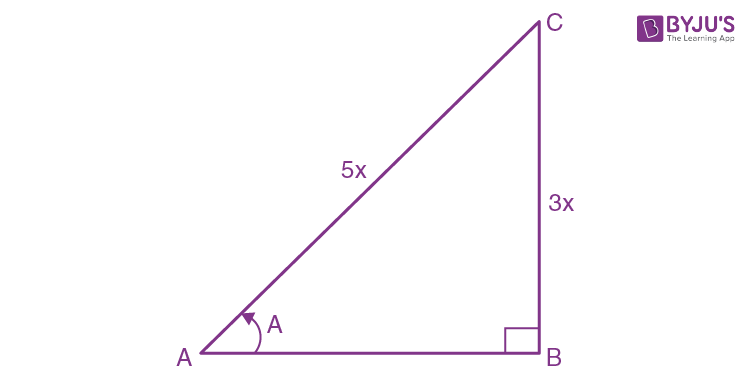Given, sin A = 3/5

⇒ perpendicular/hypotenuse = 3/5

BC/AC = 3/5

Hence,

If the length of BC is 3x, the length of AC is 5x

We have,

AB2 + BC2 = AC2 [By Pythagoras Theorem]

AB2 + (3x)2 = (5x)2

AB2 = 25x2 – 9x2

= 16x2

Taking square root on both sides, we get

AB = 4x, which is the base

Now,

(i) tan A = perpendicular/base

= 3x/4x

= 3/4

(ii) cos A = base/hypotenuse

= 4x/5x

= 4/5

6. From the following figure, find the values of:

(i) sin A

(ii) sec A

(iii) cos2 A + sin2A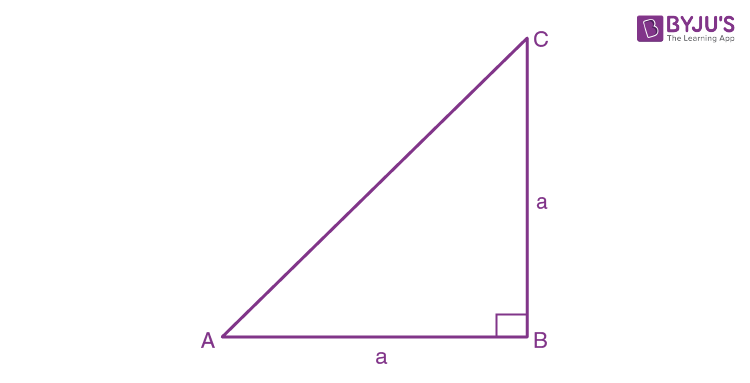Solution:

From the given figure, we have

∠ABC = 90o and AC is the hypotenuse ∆ABC

So, by Pythagoras Theorem

AC2 = AB2 + BC2

= a2 + a2

= 2a2

Taking square root on both sides, we get

AC = √2a

Now,

(i) sin A = perpendicular/hypotenuse

= BC/AB

= a/√2a

= 1/√2

(ii) sec A = hypotenuse/base

= AC/AB

= √2a/a

= √2

(iii) sin A = perpendicular/hypotenuse

= BC/AC

= a/√2a

= 1/√2

cos A = base/hypotenuse

= AB/AC

= a/√2a

= 1/√2

Hence,

cos2 A + sin2 A = (1/√2)2 + (1/√2)2

= ½ + ½

= 1

7. Given: cos A = 5/13

Evaluate: (i) (sin A – cot A)/2tan A (ii) cot A + 1/cos A

Solution:

Let’s consider the following diagram: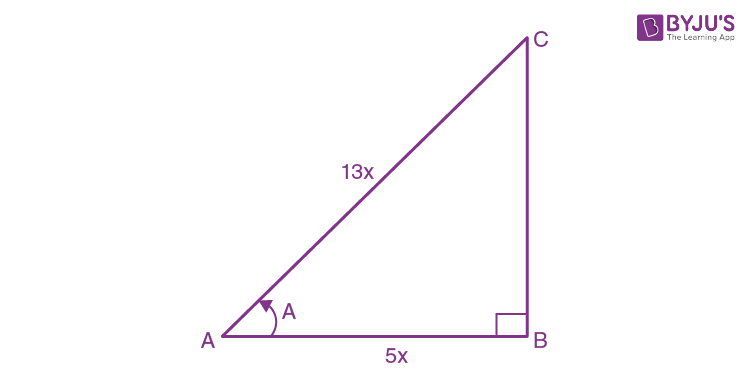Given, cos A = 5/13

⇒ base/hypotenuse = 5/13

AB/AC = 5/13

Hence,

If length of AB = 5x, the length of AC = 13x

So, by Pythagoras Theorem

AB2 + BC2 = AC2

(5x)2 + BC2 = (13x)2

BC2 = 169x2 – 25x2

= 144x2

Taking square root on both sides, we get

BC = 12x, which is the perpendicular

Now,

tan A = perpendicular/base

= 12x/5x

= 12/5

sin A = perpendicular/base

= 12x/13x

= 12/13

cot A = base/perpendicular

= 5x/12x

= 5/12

(i) (sin A – cot A)/2tan A = [(12/13) – (5/12)]/ 2(12/5)

= 79/156 x 5/24

= 395/3744

(ii) cot A + 1/cos A = 5/12 + 1/(5/13)

= 5/12 + 13/5

= 181/60

8. Given: sec A = 29/21, evaluate: sin A – 1/tan A

Solution:

Let’s consider the diagram below: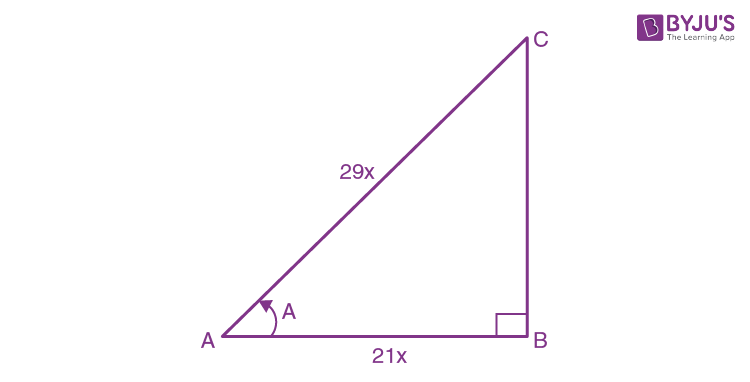Given, sec A = 29/21

⇒ hypotenuse/base = 29/21

AC/AB = 29/21

Hence,

If length of AB = 21x, the length of AC = 29x

So, by Pythagoras Theorem

AB2 + BC2 = AC2

(21x)2 + BC2 = (29x)2

BC2 = 841x2 – 441x2

= 400x2

Taking square root on both sides, we get

BC = 20x, which is the perpendicular

Now,

sin A = perpendicular/hypotenuse

= 20x/29x

= 20/29

tan A = perpendicular/base

= 20x/21x

= 20/21

Therefore,

sin A – 1/tan A = 20/29 – 1/(20/21)

= 20/29 – 21/20

= – 209/580

9. Given: tan A = 4/3, find: cosec A/(cot A – sec A)

Solution:

Let’s consider the diagram below: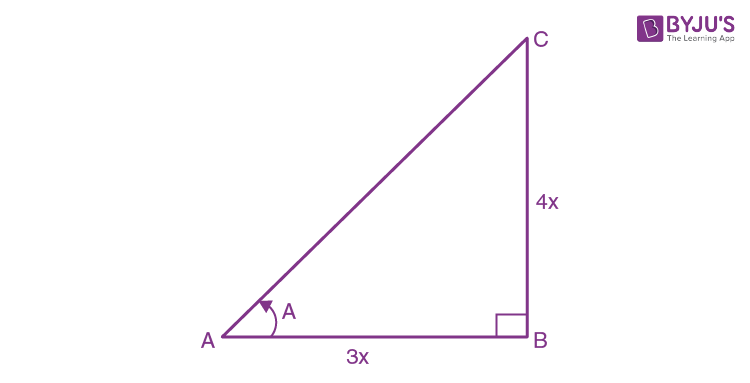Given, tan A = 4/3

⇒ perpendicular/base = 4/3

BC/AB = 4/3

Hence,

If length of AB = 3x, the length of BC = 4x

So, by Pythagoras Theorem

AB2 + BC2 = AC2

(3x)2 + (4x)2 = AC2

AC2 = 9x2 + 16x2

= 25x2

Taking square root on both sides, we get

AC = 5x, which is the hypotenuse

Now,

sec A = hypotenuse/base

= AC/AB

= 5x/3x

= 5/3

cot A = base/perpendicular

= AB/BC

= 3x/4x

= ¾

cosec A = hypotenuse/perpendicular

= AC/BC

= 5x/4x

= 5/x

Therefore,

cosec A/(cot A – sec A) = (5/4)/(3/4 – 5/3)

= (5/4)/(-11/12)

= – 60/44

= – 15/11

10. Given: 4 cot A = 3, find;

(i) sin A

(ii) sec A

(iii) cosec2 A – cot2A.

Solution:

Let’s consider the diagram below:Given, 4 cot A = 3

cot A = 3/4

⇒ base/perpendicular = 4/3

AB/BC = 3/4

Hence,

If length of AB = 3x, the length of BC = 4x

So, by Pythagoras Theorem

AB2 + BC2 = AC2

(3x)2 + (4x)2 = AC2

AC2 = 9x2 + 16x2

= 25x2

Taking square root on both sides, we get

AC = 5x, which is the hypotenuse

Now,

(i) sin A = perpendicular/hypotenuse

= 4x/5x

= 4/5

(ii) sec A = hypotenuse/base

= AC/AB

= 5x/3x

= 5/3

(iii) cosec A = hypotenuse/perpendicular

= AC/BC

= 5x/4x

= 5/4

cot A = 3/4

Hence,

cosec2 A – cot2 A = (5/4)2 – (3/4)2

= (25 – 9)/16

= 16/16

= 1

Exercise 22(B)

1. From the following figure, find:

(i) y

(ii) sin xo

(iii) (sec xo – tan xo) (sec xo + tan xo)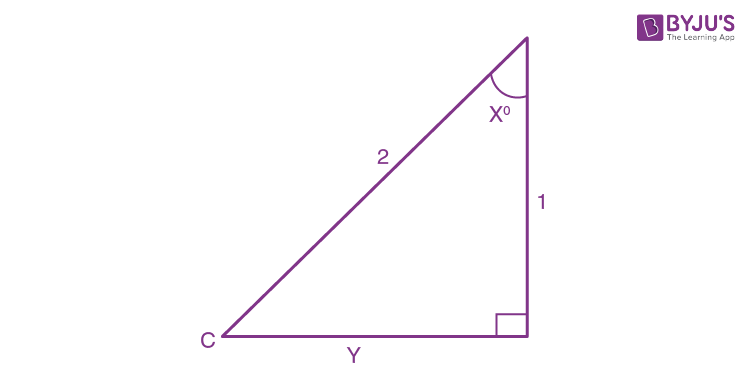Solution:

In the given figure,

(i) As it’s a right-angled triangle, so using Pythagorean Theorem

22 = y2 + 12

y2 = 22 – 12

= 4 – 1

= 3

Taking square root on both sides, we get

y = √3

(ii) sin xo = perpendicular/hypotenuse

= √3/2

(iii) tan xo = perpendicular/base

= √3

sec xo = hypotenuse/base

= 2

Therefore,

(sec xo – tan xo) (sec xo + tan xo) = (2 – √3)(2 + √3)

= 4 – √3

= 1

2. Use the given figure to find:

(i) sin xo

(ii) cos yo

(iii) 3 tan xo – 2 sin yo + 4 cos yo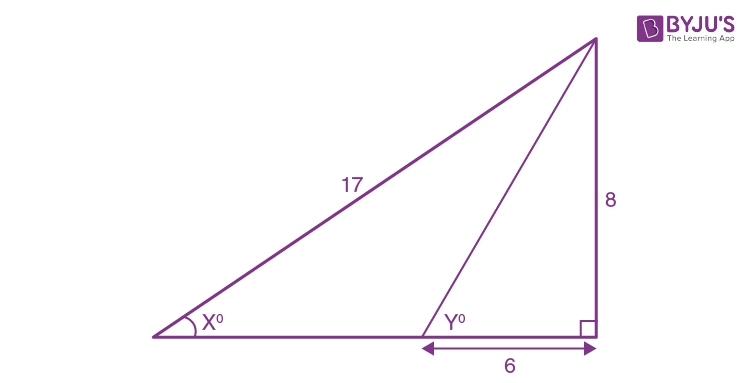Solution:

Let’s consider the given figure,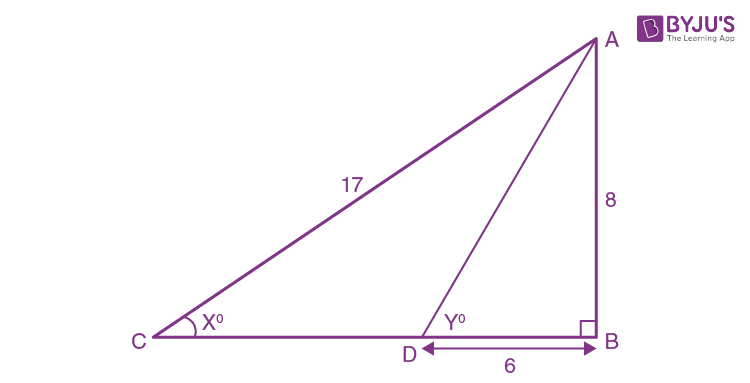As the triangle is a right-angled triangle, so using Pythagorean Theorem

AD2 = 82 + 62

= 64 + 36

= 100

Taking square root on both sides, we get

Also, by Pythagorean Theorem

BC2 = AC2 – AB2

= 172 – 82

= 289 – 64

= 225

Taking square root on both sides, we get

BC = 15

Now,

(i) sin xo = perpendicular/hypotenuse

= 8/17

(ii) cos yo = base/hypotenuse

= 6/10

= 3/5

(iii) sin yo = perpendicular/base

= 8/10

= 4/5

And,

cos yo = 6/10

= 3/5

So,

tan xo = perpendicular/base

= AB/BC

= 8/15

Therefore,

3 tan xo – 2 sin yo + 4 cos yo

= 3(8/15) – 2(4/5) + 4(3/5)

= 8/5 – 8/5 + 12/5

= 12/5

3. In the diagram, given below, triangle ABC is right-angled at B and BD is perpendicular to AC. Find:

(i) cos ∠DBC (ii) cot ∠DBA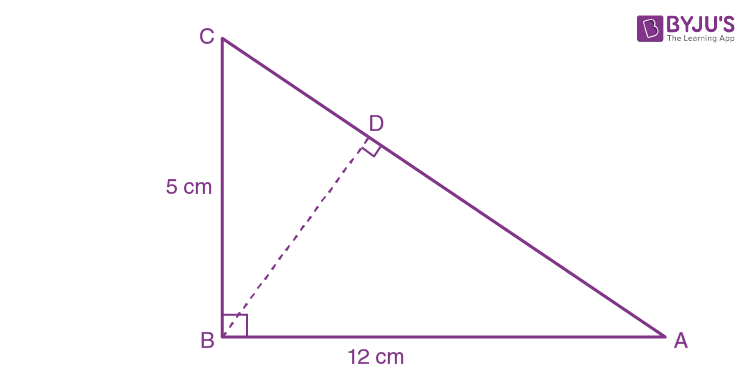Solution:

Let’s consider the given figure,

As the triangle is a right-angled triangle, so using Pythagorean Theorem

AC2 = 52 + 122

= 25 + 144

= 169

Taking square root on both sides, we get

AC = 13

In ∆CBD and ∆CBA,

∠C is common to both the triangles

∠CDB = ∠CBA = 90o

Hence, ∠CBD = ∠CAB

Thus, ∆CBD and ∆CBA are similar triangles according to AAA criterion

So, we have

AC/BC = AB/BD

13/5 = 12/BD

BD = 60/13

Now,

(i) cos ∠DBC = base/hypotenuse

= BD/BC

= (60/13)/5

= 12/13

(ii) cot ∠DBA = base/perpendicular

= BD/AB

= (60/13)/12

= 5/13

4. In the given figure, triangle ABC is right-angled at B. D is the foot of the perpendicular from B to AC. Given that BC = 3 cm and AB = 4 cm. Find:

(i) tan ∠DBC

(ii) sin ∠DBA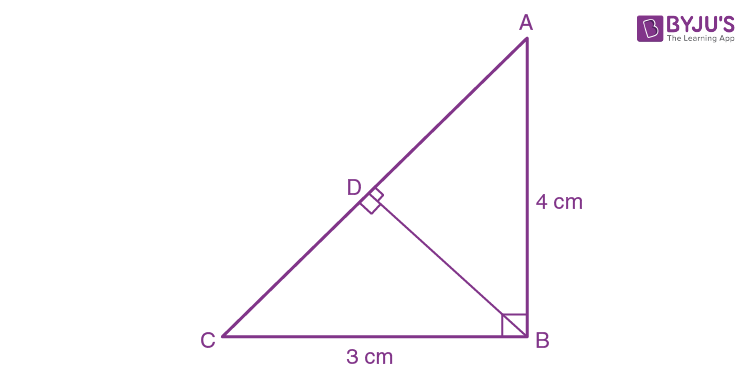Solution:

Considering the given figure, we have

A right-angled triangle ABC, so by using Pythagorean Theorem we have

AC2 = BC2 + AB2

= 42 + 32

= 16 + 9

= 25

Taking square root on both sides, we get

AC = 5

In ∆CBD and ∆CAB, we have

∠BCD = ∠ACB (Common)

∠CDB = ∠CBA = 90o

Hence, ∆CBD ~ ∆CAB by AA similarity criterion

So,

AC/BC = AB/BD

5/3 = 4/BD

BD = 12/5

Now, using Pythagorean Theorem in ∆BDC

DC2 = BC2 – BD2

= 32 – (12/5)2

= 9 – 144/25

= (225 – 144)/25

= 81/25

Taking square root on both sides, we get

DC = 9/5

Therefore,

AD = AC – DC

= 5 – 9/5

= 16/5

Now,

(i) tan ∠DBC = perpendicular/ base

= DC/BD

= (9/5)/(12/5)

= 3/4

(ii) sin ∠DBA = AD/AB

= (16/5)/4

= 4/5

5. In triangle ABC, AB = AC = 15 cm and BC = 18 cm, find cos ∠ABC.

Solution:

Let’s consider the figure below: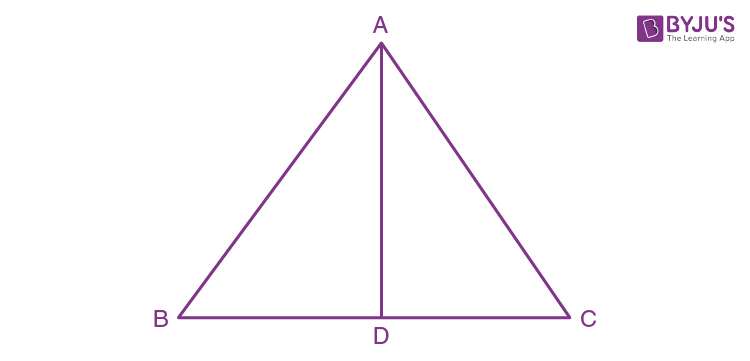In the isosceles ∆ABC, we have

AB = AC = 15 cm

BC = 18 cm

Now, the perpendicular drawn from angle A to its opposite BC divides its into two equal parts

i.e., BD = DC = 9cm

Hence,

cos ABC = base/hypotenuse

= BD/AB

= 9/15

= 3/5

6. In the figure given below, ABC is an isosceles triangle with BC = 8 cm and AB = AC = 5 cm. Find:

(i) sin B

(ii) tan C

(iii) sin2 B + cos2

(iv) tan C – cot B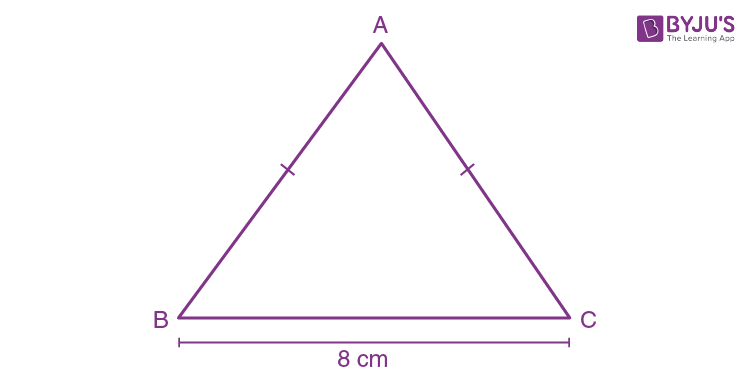Solution:

Let’s consider the figure below: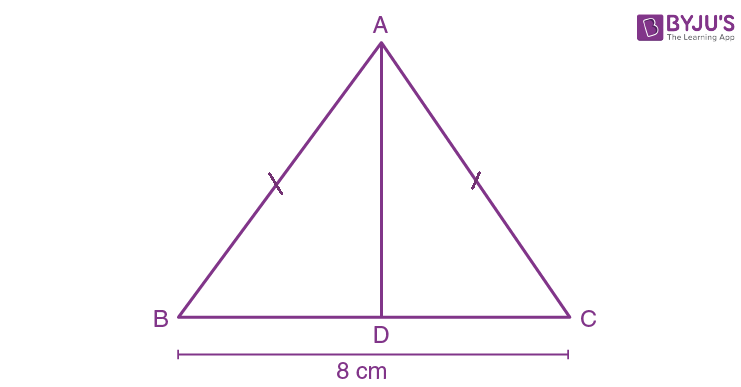In the isosceles ∆ABC, we have

AB = AC = 5 cm

BC = 8 cm

Now, the perpendicular drawn from angle A to its opposite BC divides its into two equal parts

i.e., BD = DC = 4cm

As, ∠ADB = 90o in ∆ABD, we have

AB2 = AD2 + BD2

AD2 = AB2 – BD2

= 52 – 42

= 25 – 16

= 9

Taking square root on both sides, we get

Now,

(i) sin B = AD/AB

= 3/5

(ii) tan C = AD/DC

= 3/4

(iii) sin B = AD/AB

= 3/5

cos B = BD/AB

= 4/5

Hence,

sin2 B + cos2 B = (3/5)2 + (4/5)2

= 9/25 + 16/25

= 25/25

= 1

(iv) tan C = AD/DC

= ¾

cot B = BD/AD

= 4/3

Hence,

tan C – cot B = ¾ – 4/3

= (9 – 16)/12

= -7/12

7. In triangle ABC; ∠ABC = 90o, ∠CAB = xo, tan xo = ¾ and BC = 15 cm. Find the measures of AB and AC.

Solution:

Let’s consider the figure below: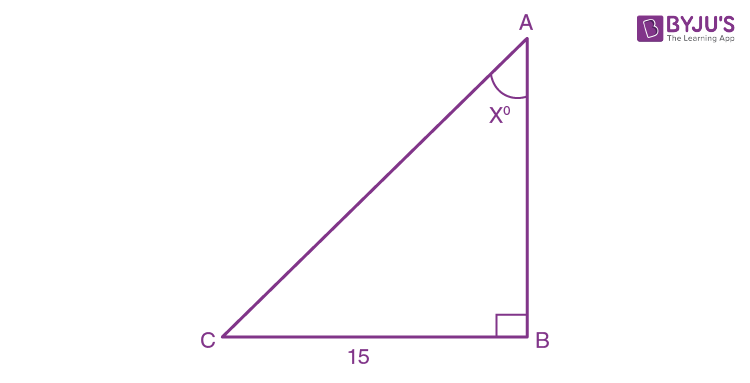Given, tan xo = ¾

⇒ perpendicular/base = ¾

BC/AB = ¾

Hence,

If length of base AB = 4x, the length of perpendicular BC = 3x

So, by Pythagoras Theorem

BC2 + AB2 = AC2

(3x)2 + (4x)2 = AC2

AC2 = 9x2 + 16x2

= 25x2

Taking square root on both sides, we get

AC = 5x, which is the hypotenuse

Now, we have

BC = 15

⇒ 3x = 15

x = 5

Therefore, AB = 4x = 4(5) = 20 cm

And, AC = 5x = 5 × 5 = 25 cm

8. Using the measurements given in the following figure:

(i) Find the value of sin Ø and tan θ

(ii) Write an expression for AD in terms of θ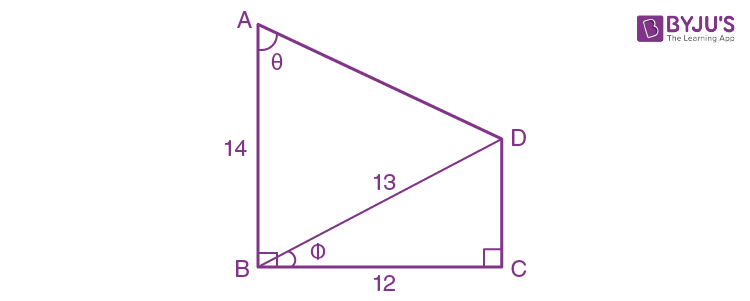Solution:

Let’s consider the figure below: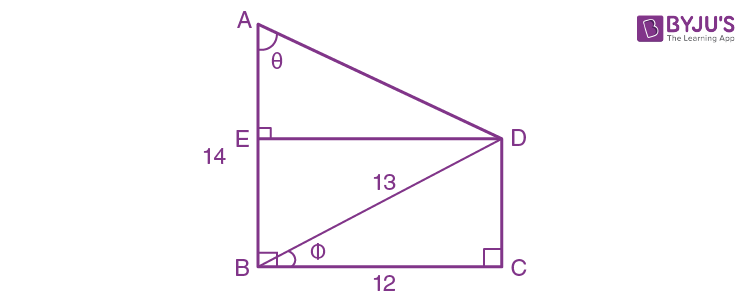Constructing a perpendicular from D to the side AB at point E which makes BCDE a rectangle.

Now, in right angled ∆BCD using Pythagorean Theorem, we have

BD2 = BC2 + CD2 [As AB is the hypotenuse]

CD2 = BD2 – BC2

CD2 = 132 – 122

= 169 – 144

= 25

Taking square root on both sides, we get

CD = 5

As BCDE is a rectangle,

ED = 12 cm, EB = 5 cm and AE = (14 – 5) cm = 9 cm

Now,

(i) sin Ø = CD/BD

= 5/13

tan θ = ED/AE

= 12/9

= 4/3

(ii) sec θ = AD/AE

AD = 9 sec θ

Or

cosec θ = AD/ED

AD = 12cosec θ

9. In the given figure:

BC = 15 cm and sin B = 4/5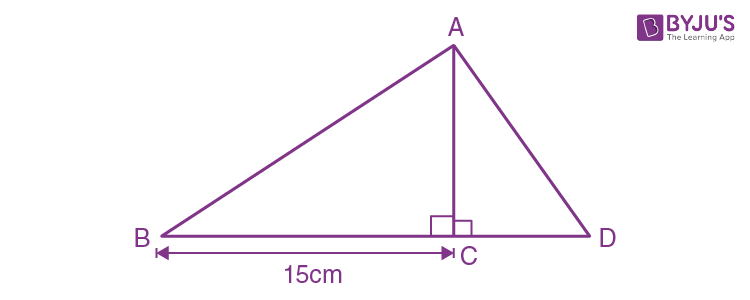(i) Calculate the measure of AB and AC.

(ii) Now, if tan ∠ADC = 1; calculate the measures of CD and AD.

Also, show that: tan2B – 1/cos2 B = -1

Solution:

Given, BC = 15 cm and sin B = 4/5

⇒ Perpendicular/hypotenuse = AC/AB

= 4/5

Hence, if the length of perpendicular is 4x, the length of hypotenuse will be 5x

In right triangle ABC, we have

BC2 + AC2 = AB2 [By Pythagoras Theorem]

BC2 = AB2 – AC2

= (5x)2 – (4x)2

= 25x2 – 16x2

= 9x2

Taking square root on both sides, we get

BC = 3x

Now, as BC = 15 (given)

3x = 15

x = 15/3

x = 5

(i) AC = 4x

= 4(5)

= 20 cm

And,

AB = 5x

= 5(5)

= 25 cm

(ii) Given,

tan ∠ADC = 1

perpendicular/base = AC/CD

= 1/1

Hence,

If length of perpendicular is x, then the length of hypotenuse will be x

And, we have

AC2 + CD2 = AD2 [Using Pythagoras Theorem]

x2 + x2 = AD2

Taking square root on both sides, we get

Now,

AC = 20 ⇒x = 20

So,

AD = √2x = √2(20) = 20√2 cm

And,

CD = 20 cm

Hence,

tan B = AC/BC

= 20/15

= 4/3

cos B = BC/AB

= 15/25

= 3/5

Thus,

tan2 B – 1/cos2 B = (4/3)2 – 1/(3/5)2

= 16/9 – 1/(9/25)

= 16/9 – 25/9

= – 9/9

= -1

10. If sin A + cosec A = 2;

Find the value of sin2 A + cosec2 A.

Solution:

Given, sin A + cosec A = 2

On squaring on both sides, we have

(sin A + cosec A)2 = 22

sin2 A + cosec2 A + 2sinA. cosec A = 4

sin2 A + cosec2 A + 2 = 4 [As sin A. cosec A = sin A x 1/sin A = 1]

sin2 A + cosec2 A = 4 – 2 = 2

Hence, the value of (sin2 A + cosec2 A) is 2

## Selina Solutions for Class 9 Maths Chapter 22- Trigonometrical Ratios

Chapter 22, Trigonometrical Ratios, consists of 2 exercises and the solutions given here contain answers to all the questions present in these exercises. Let us have a look at some of the topics that are being discussed in this chapter.

22.1 Introduction

22.2 Concept of perpendicular, base and hypotenuse in a right triangle

22.3 Notation of angles

22.4 Trigonometrical Ratios

22.5 Reciprocal Relations

## Selina Solutions for Class 9 Maths Chapter 22- Trigonometrical Ratios

The ratio between the lengths of a pair of two sides of a right-angled triangle is called a Trigonometrical Ratio. The three sides of a right-angled triangle give six trigonometrical ratios; namely sine, cosine, tangent, cotangent, secant, and cosecant. Here, in this chapter, students are taught about the different Trigonometrical Ratios. Read and learn Chapter 22 of Selina textbook to get to know more about Trigonometrical Ratios. Learn the Selina Solutions for Class 9 effectively to attain excellent results in the examination.